# RS Aggarwal Solutions for Class 7 Maths Chapter 1 Integers

RS Aggarwal Solutions for Class 7 Maths Chapter 1 – Integers are available here. These exercises are formulated by our expert tutors in order to assist you with your exam preparation to attain good marks in Maths. Students who wish to score good marks in Maths practise RS Aggarwal Class 7 Solutions. This book is one of the top materials when it comes to providing a question bank to practice from.

The main aim is to help students understand and crack these problems. We at BYJU’S have prepared the RS Aggarwal Solutions for Class 7 Maths Chapter 1 wherein problems are solved step by step with detailed explanations. Download pdf of Class 7 Chapter 1 in their respective links.

## Download PDF of RS Aggarwal Solutions for Class 7 Maths Chapter 1 – Integers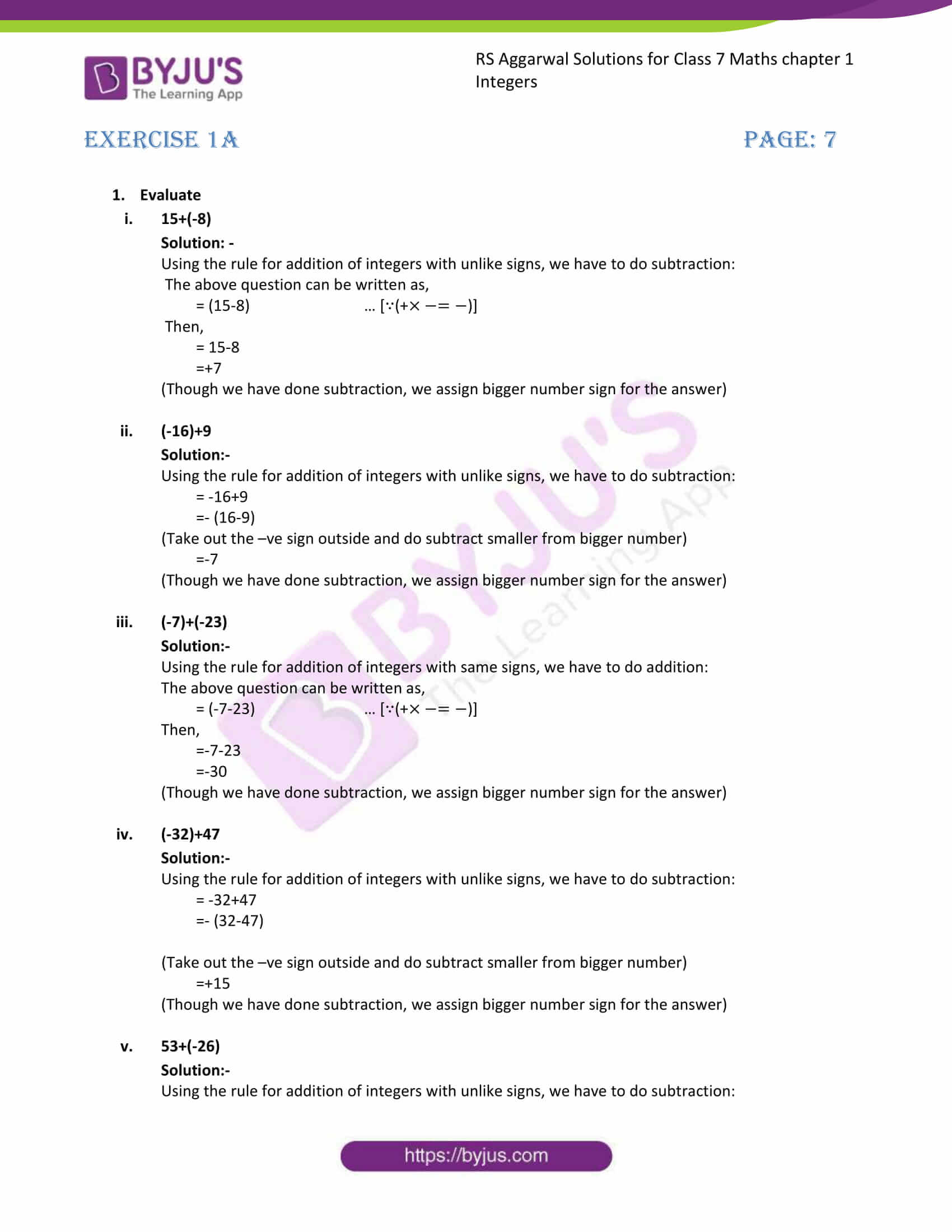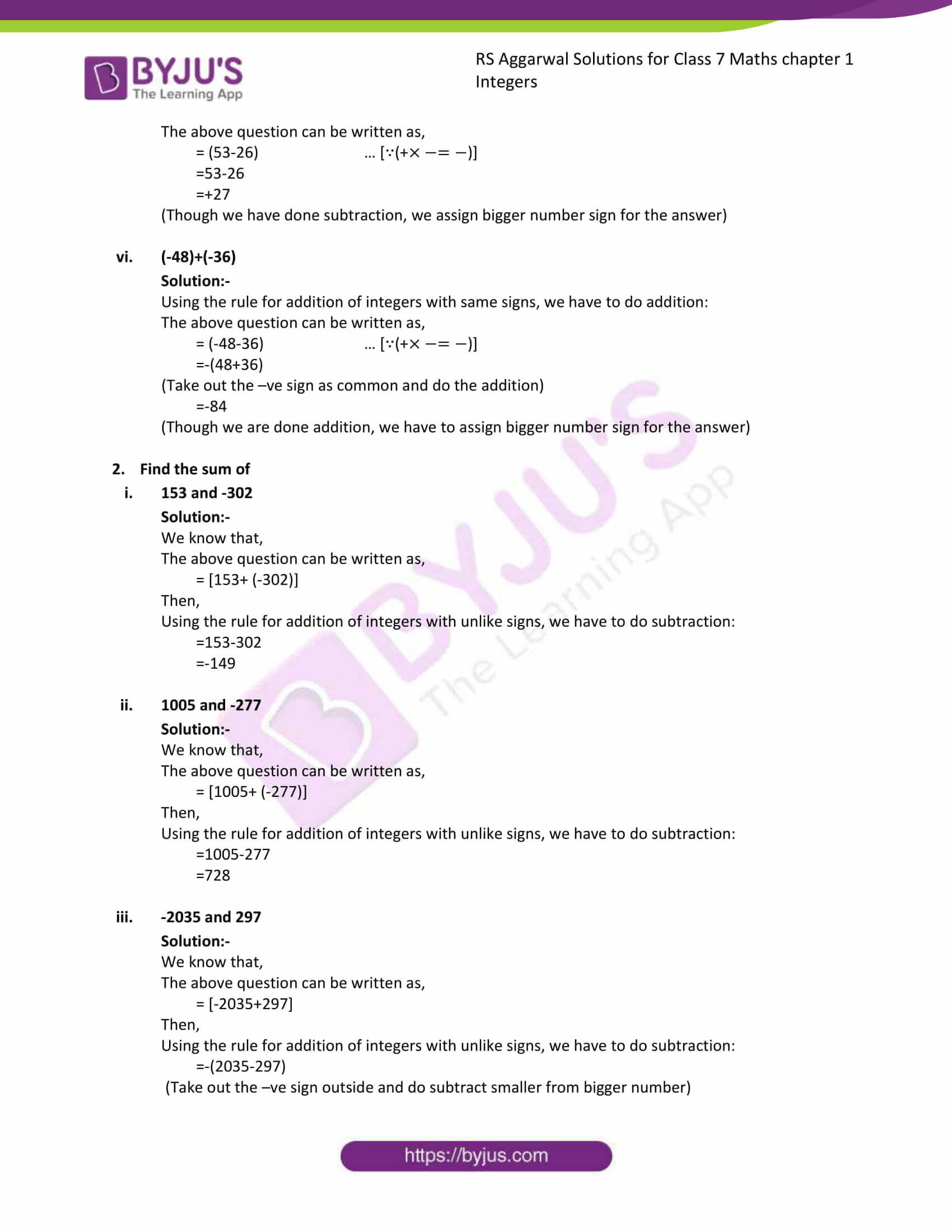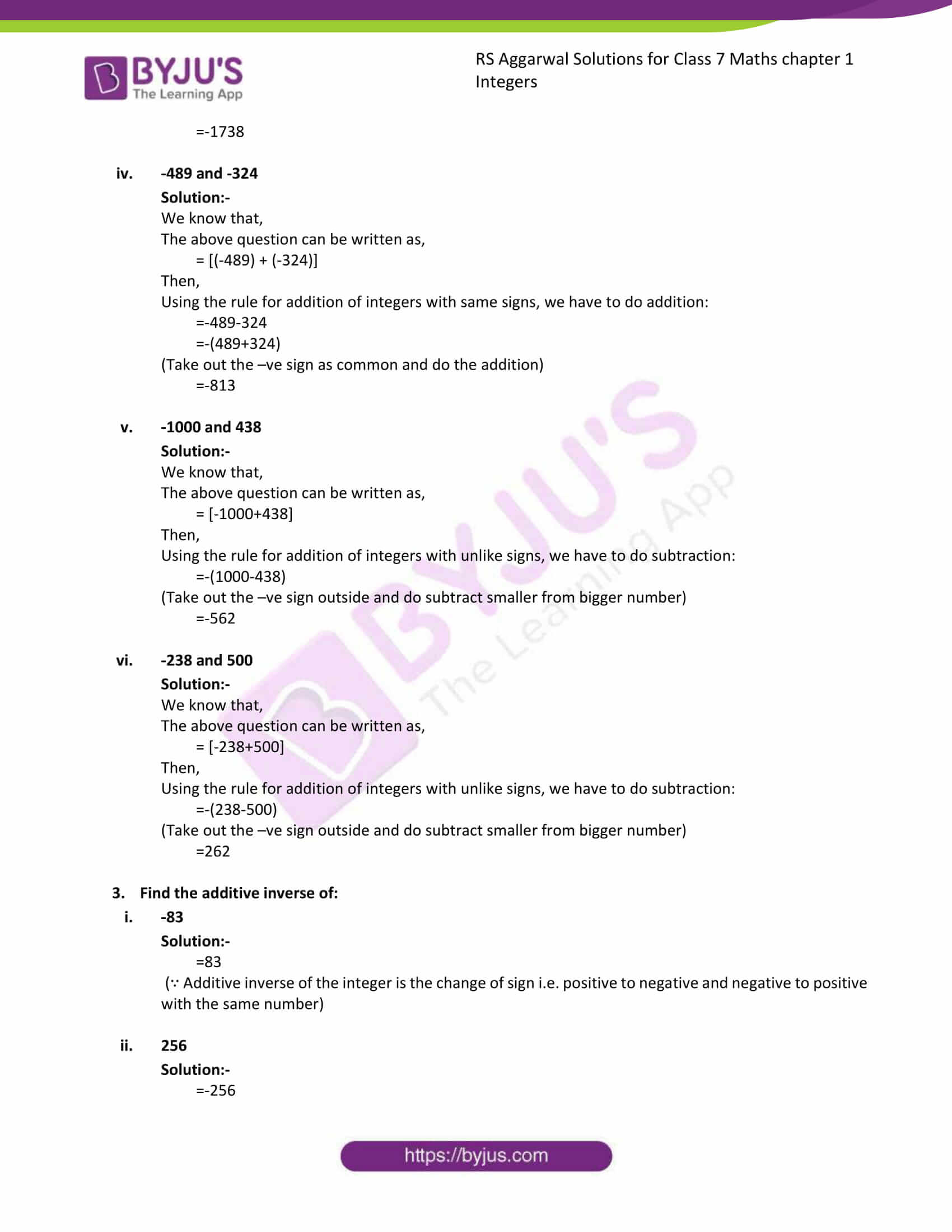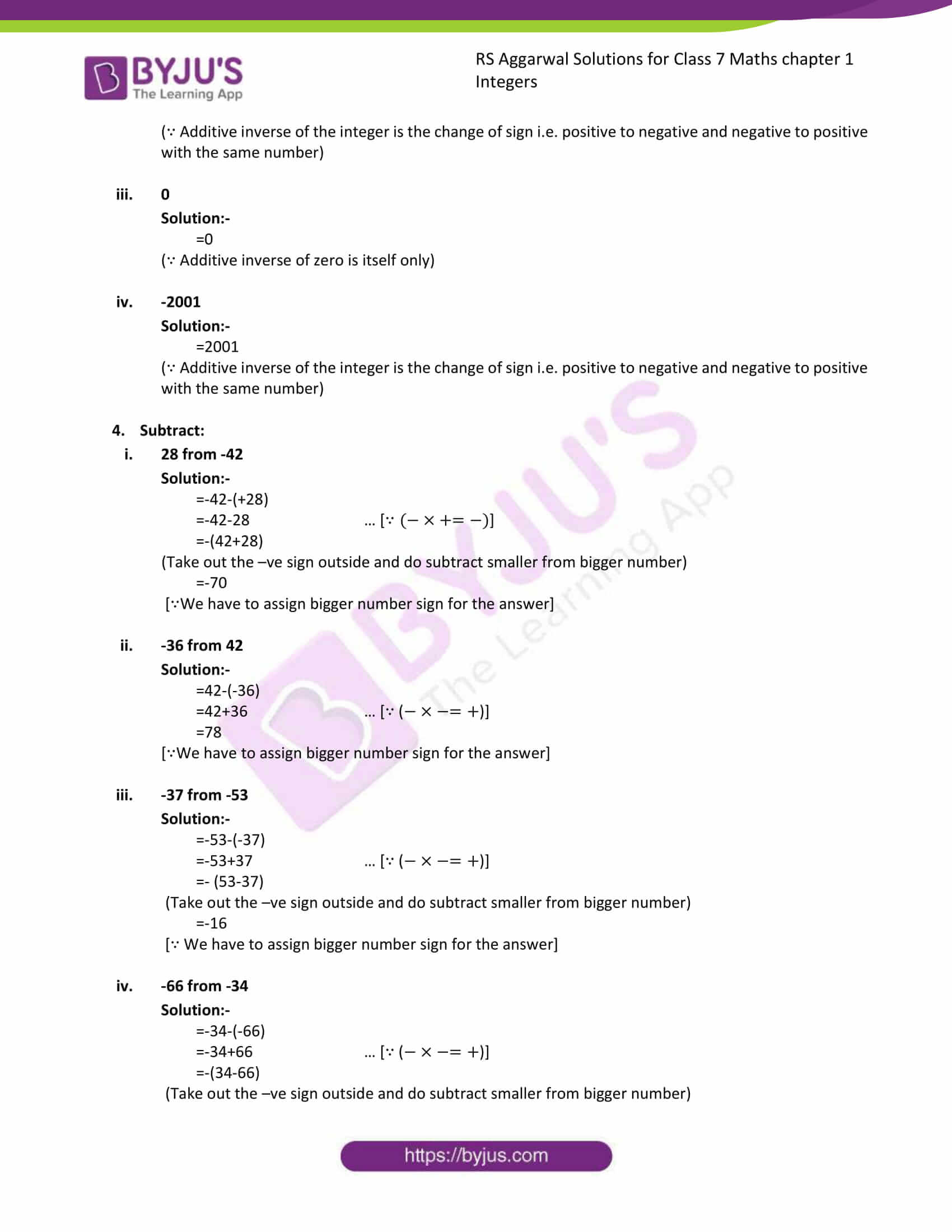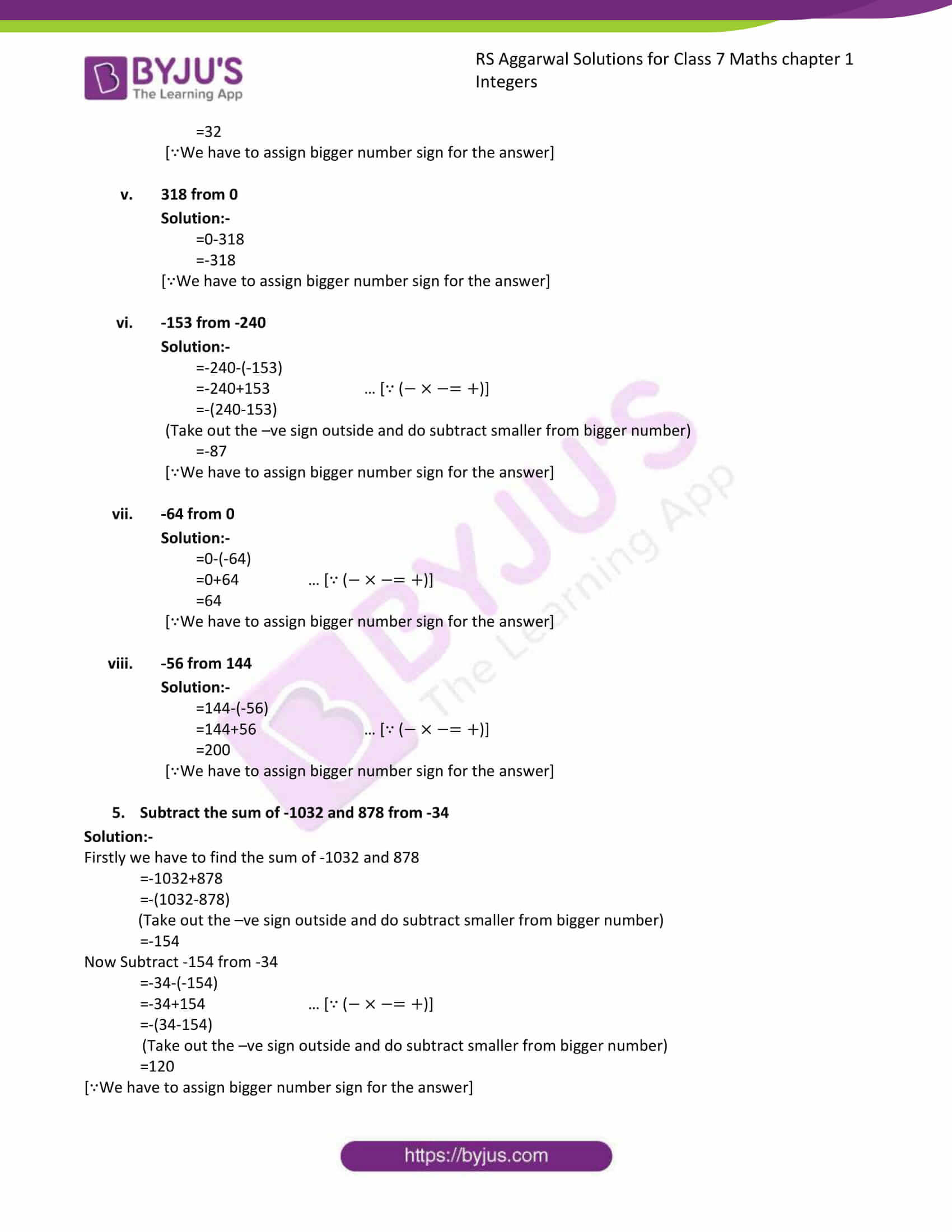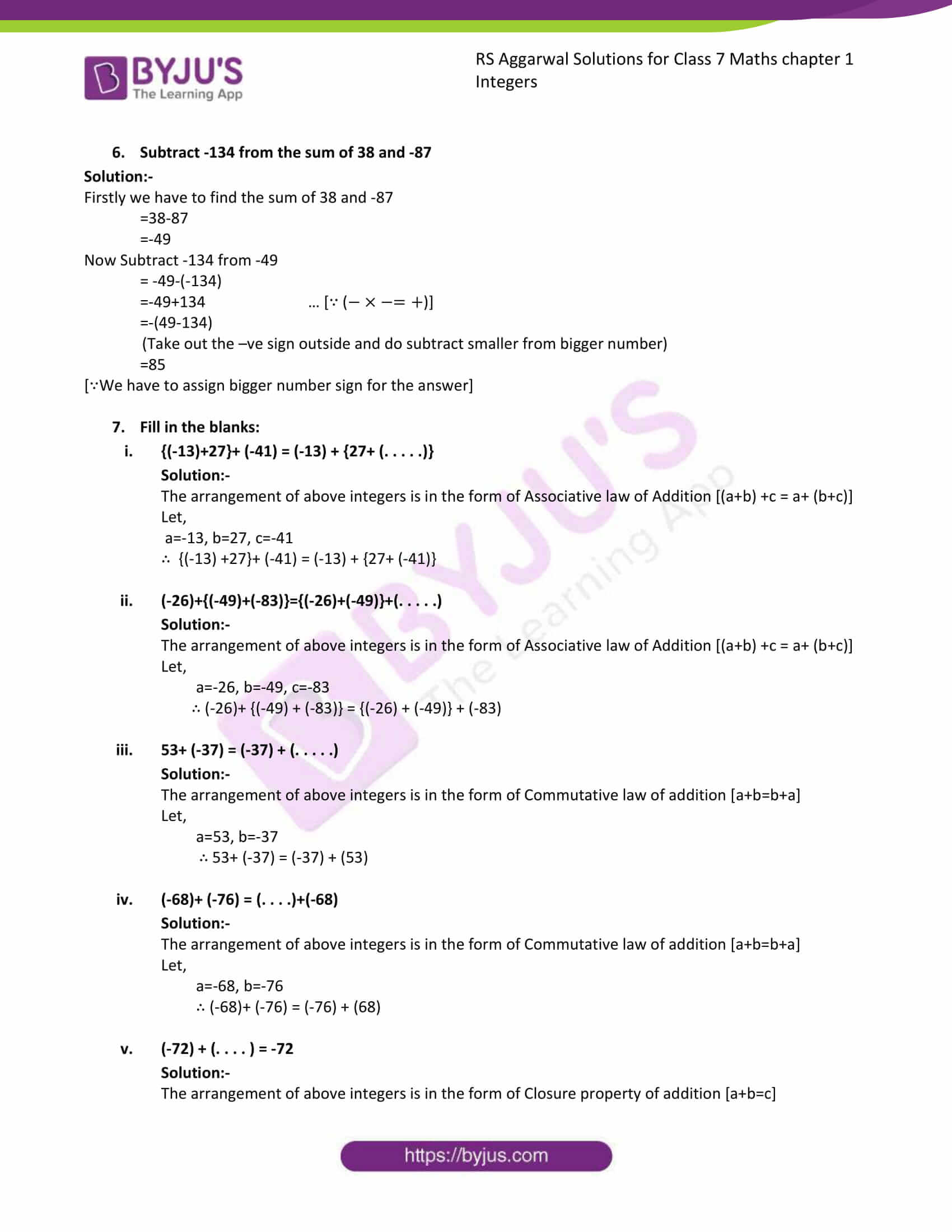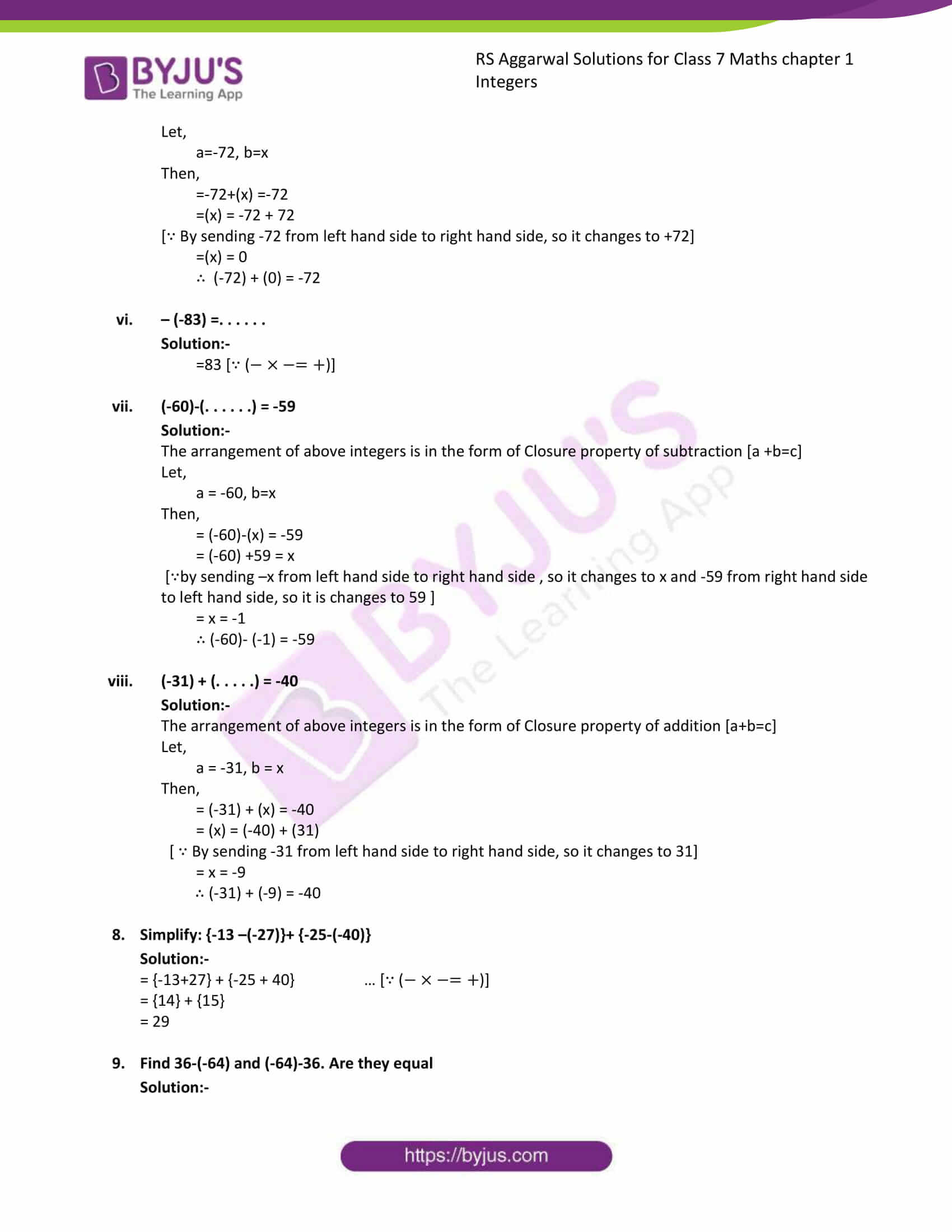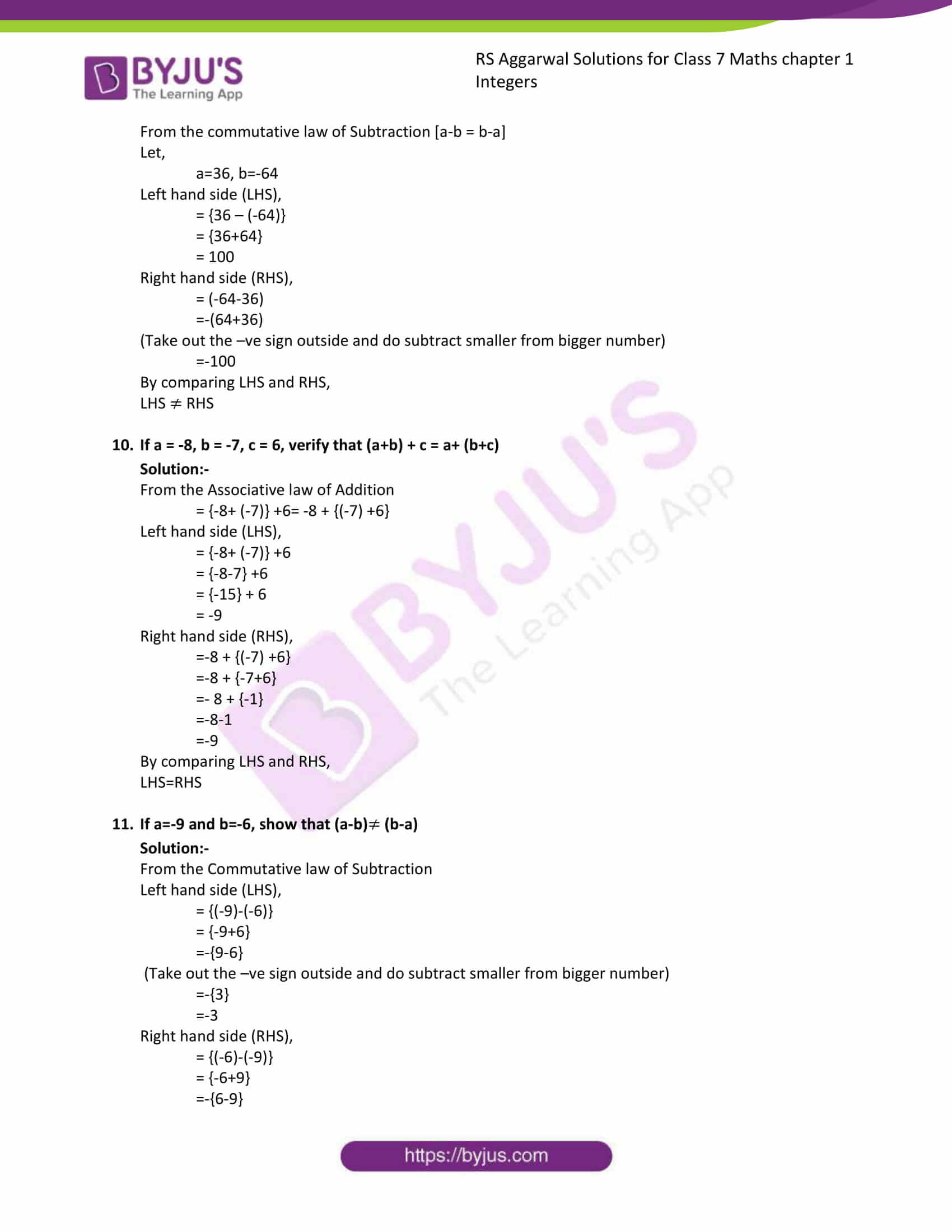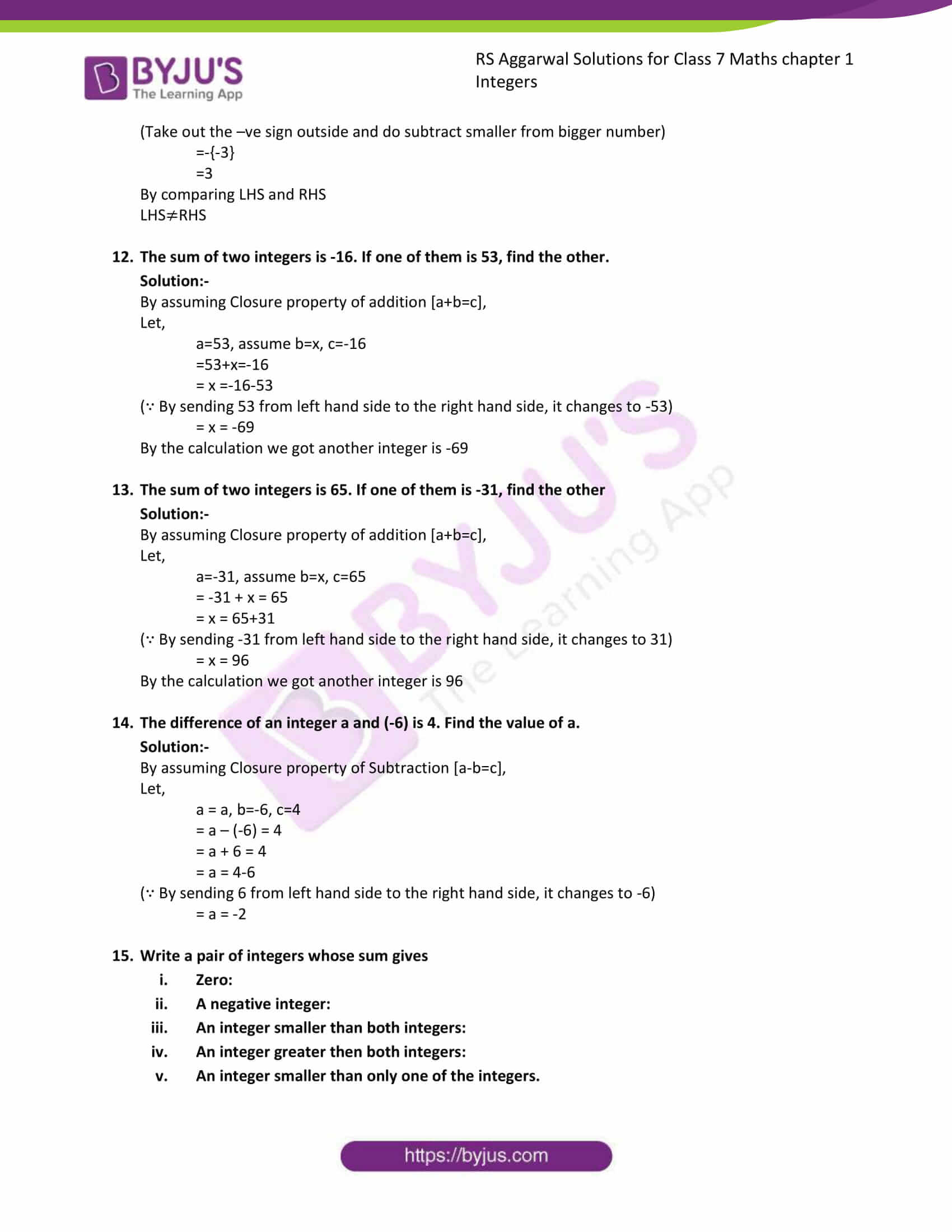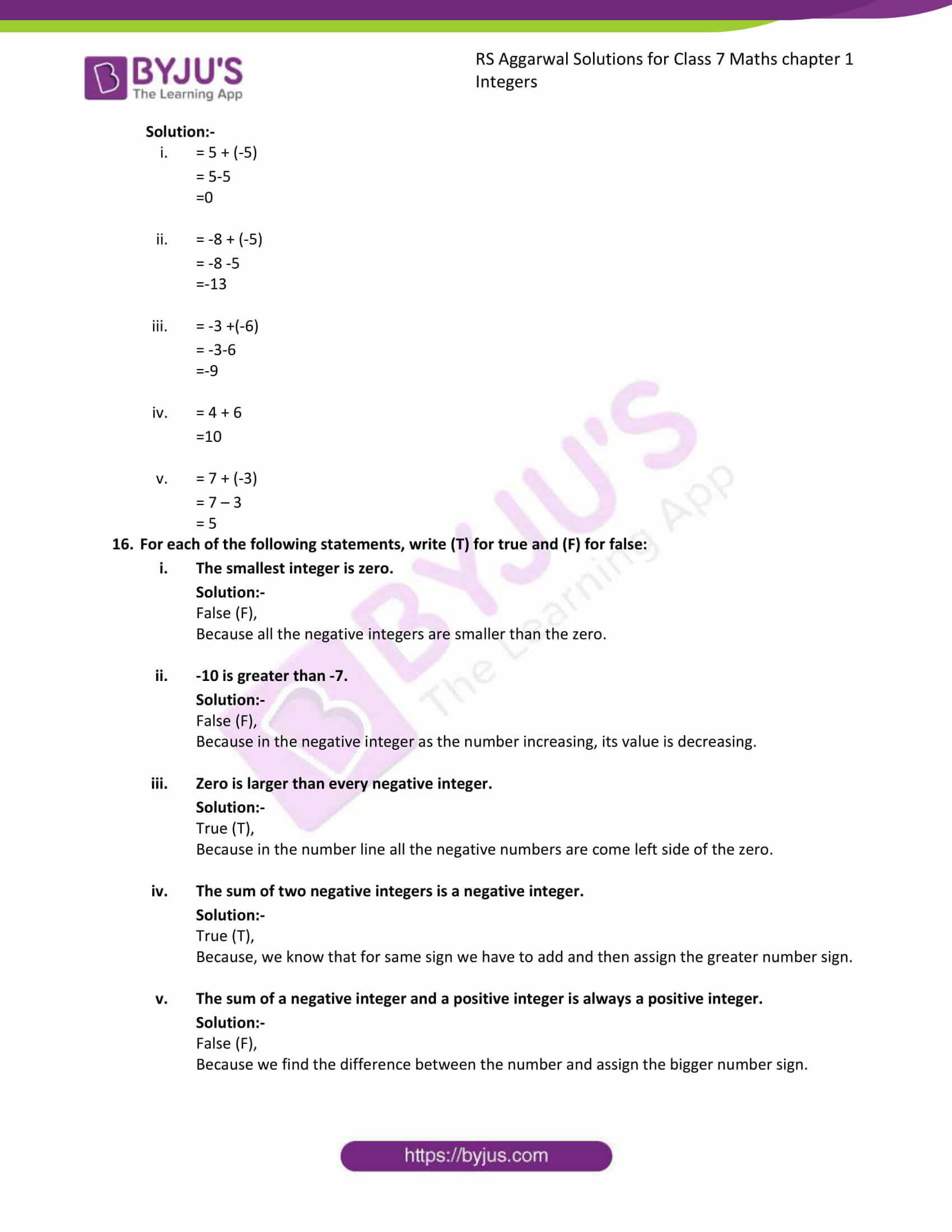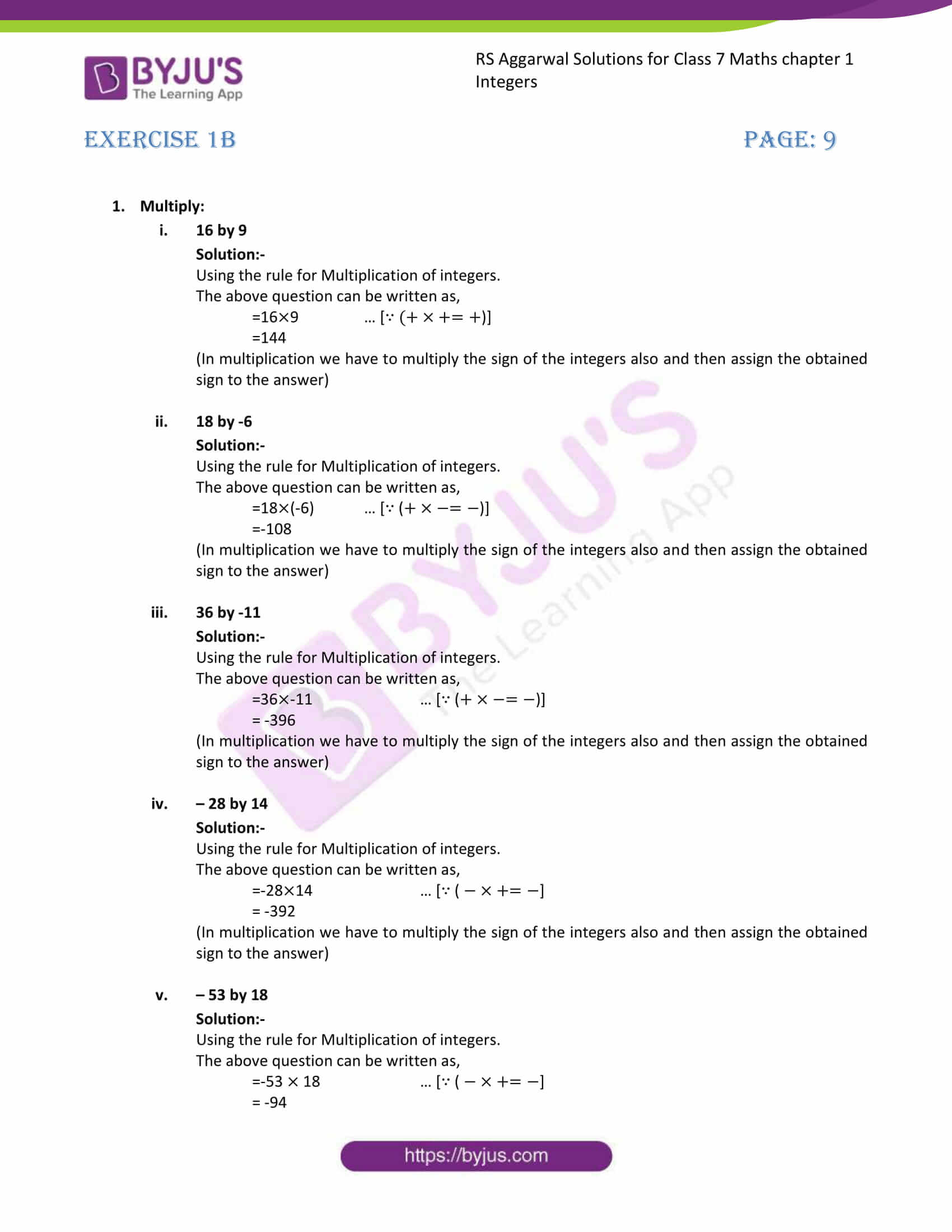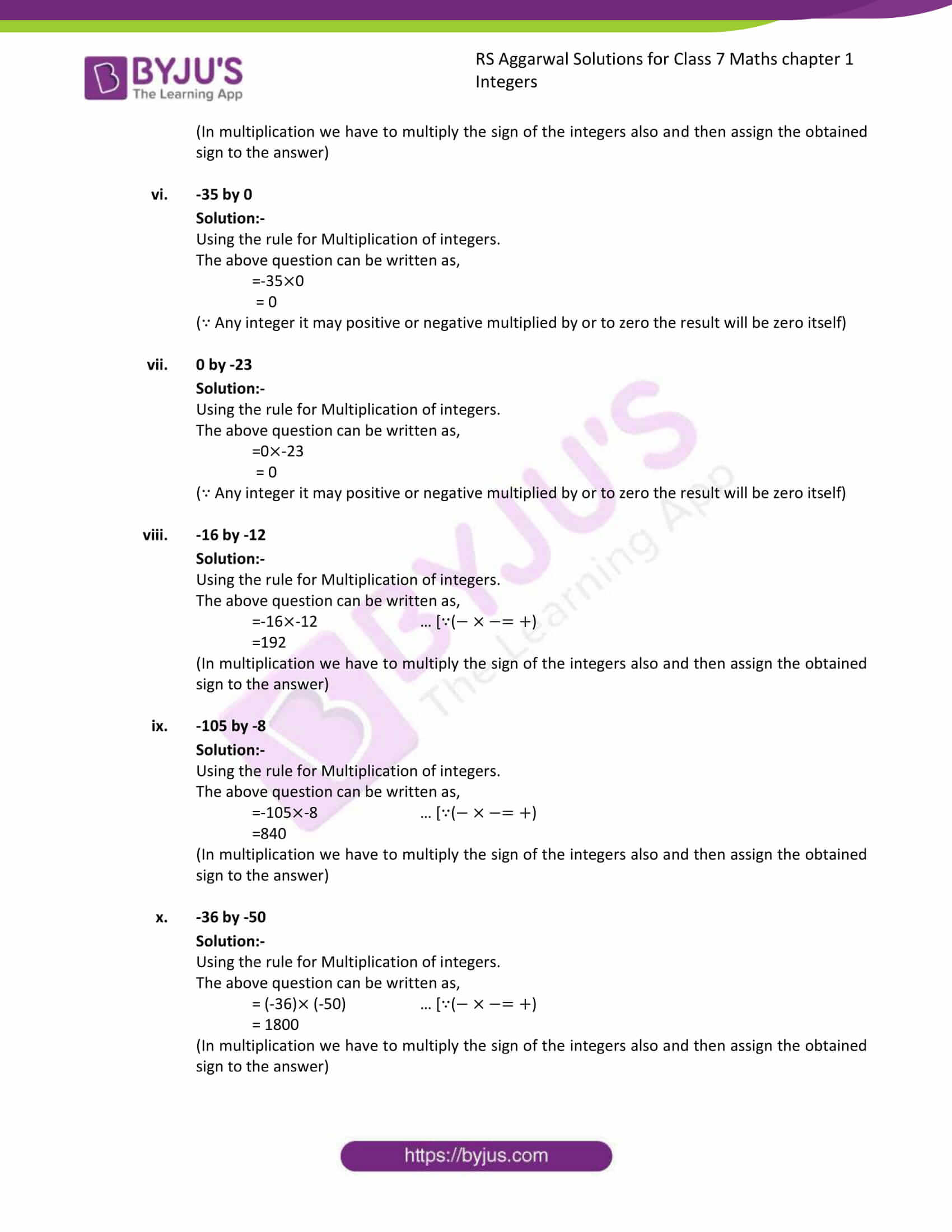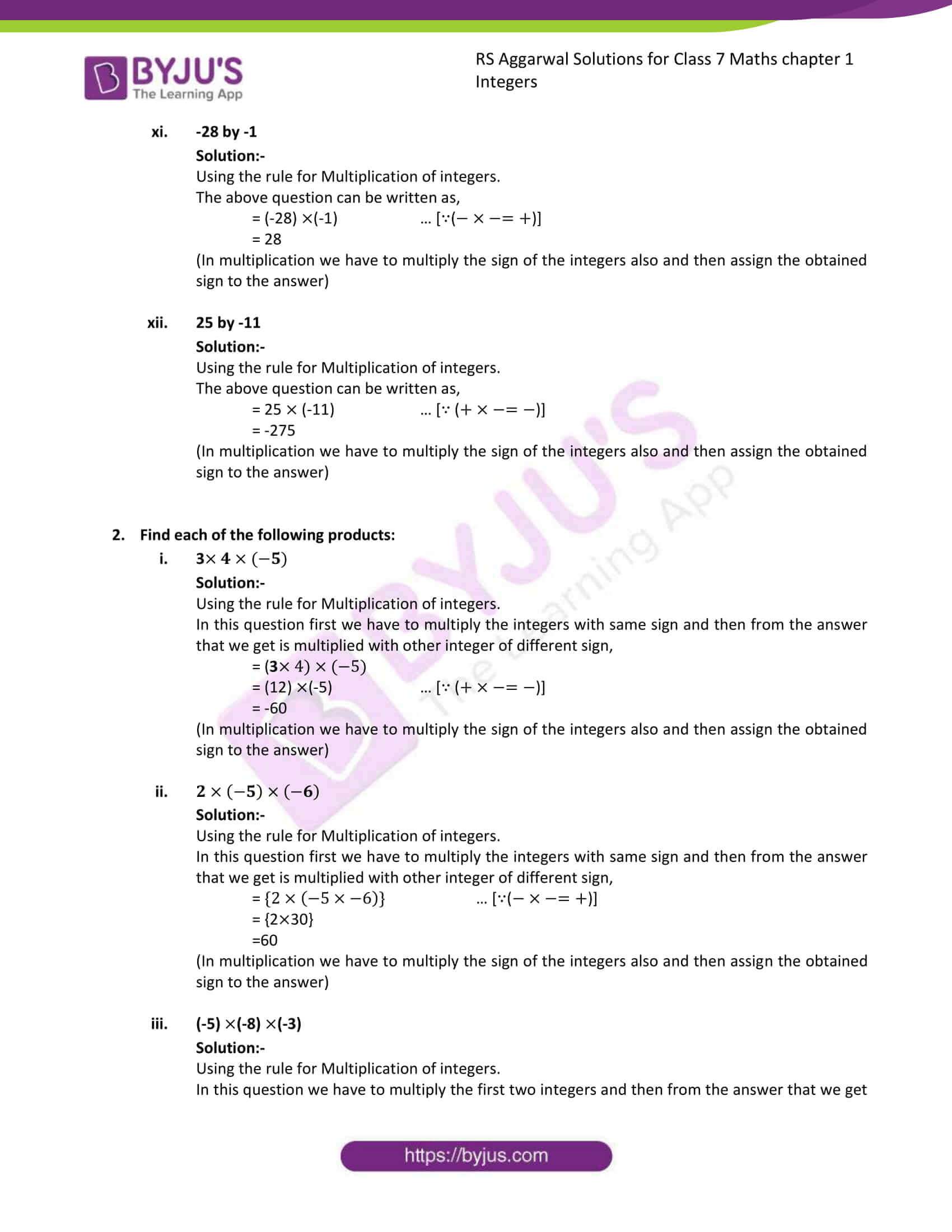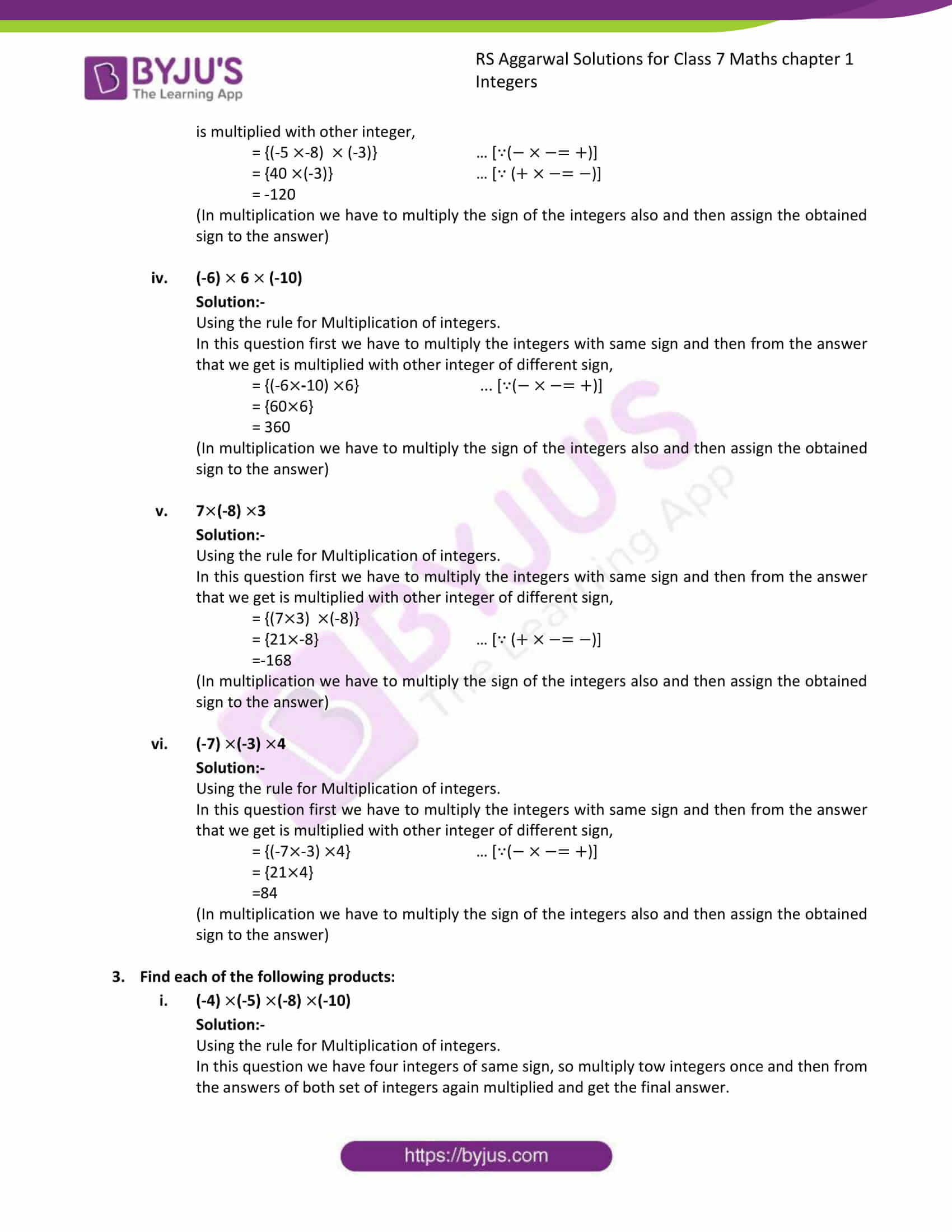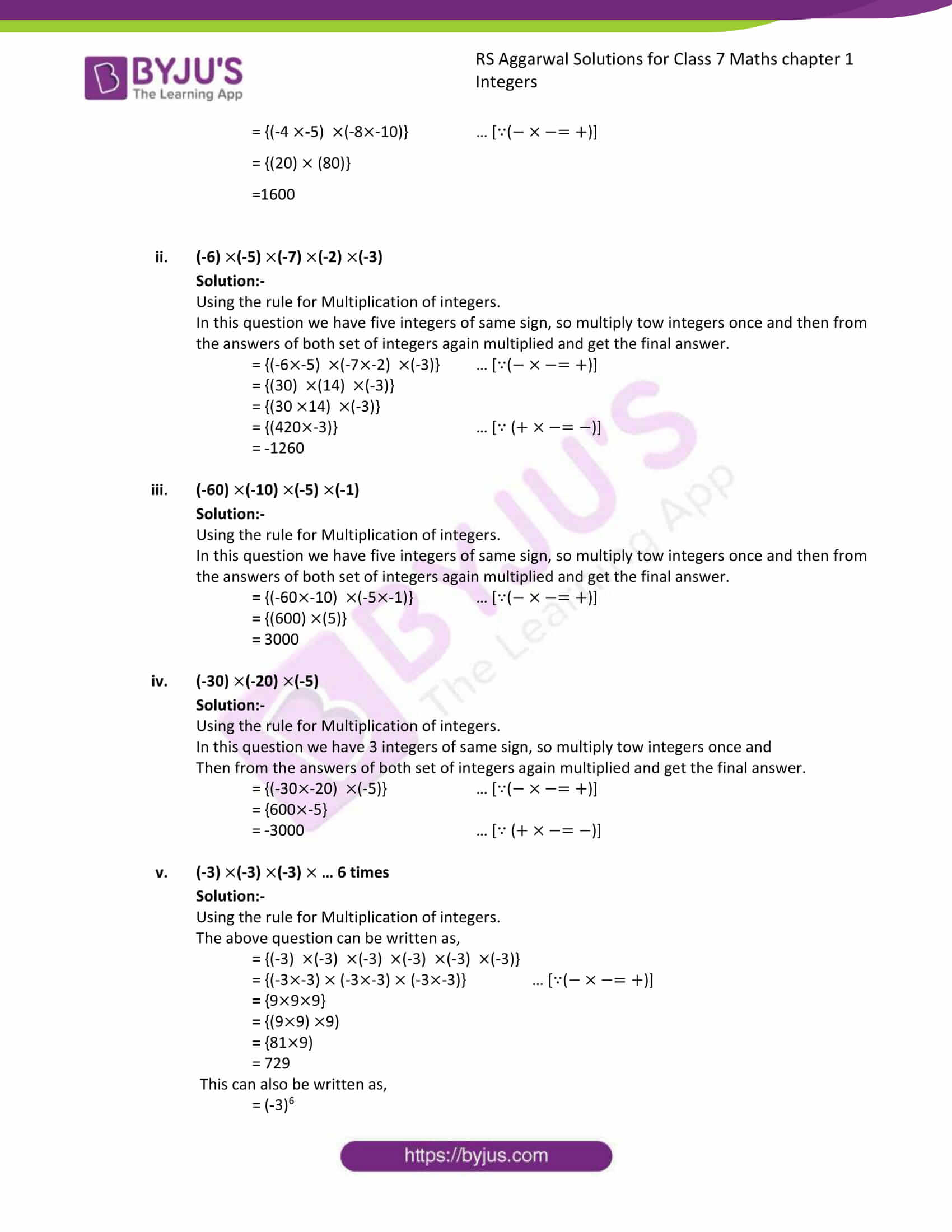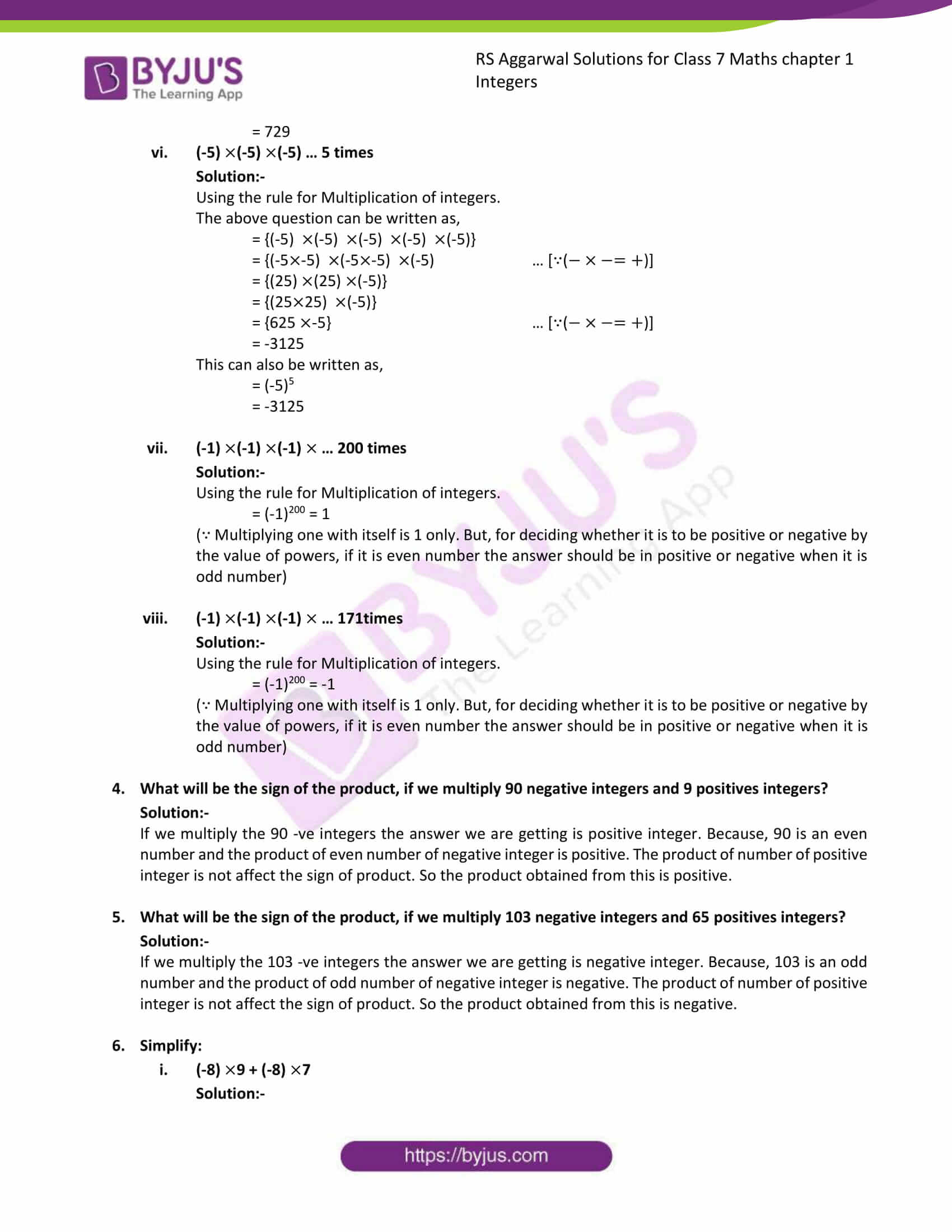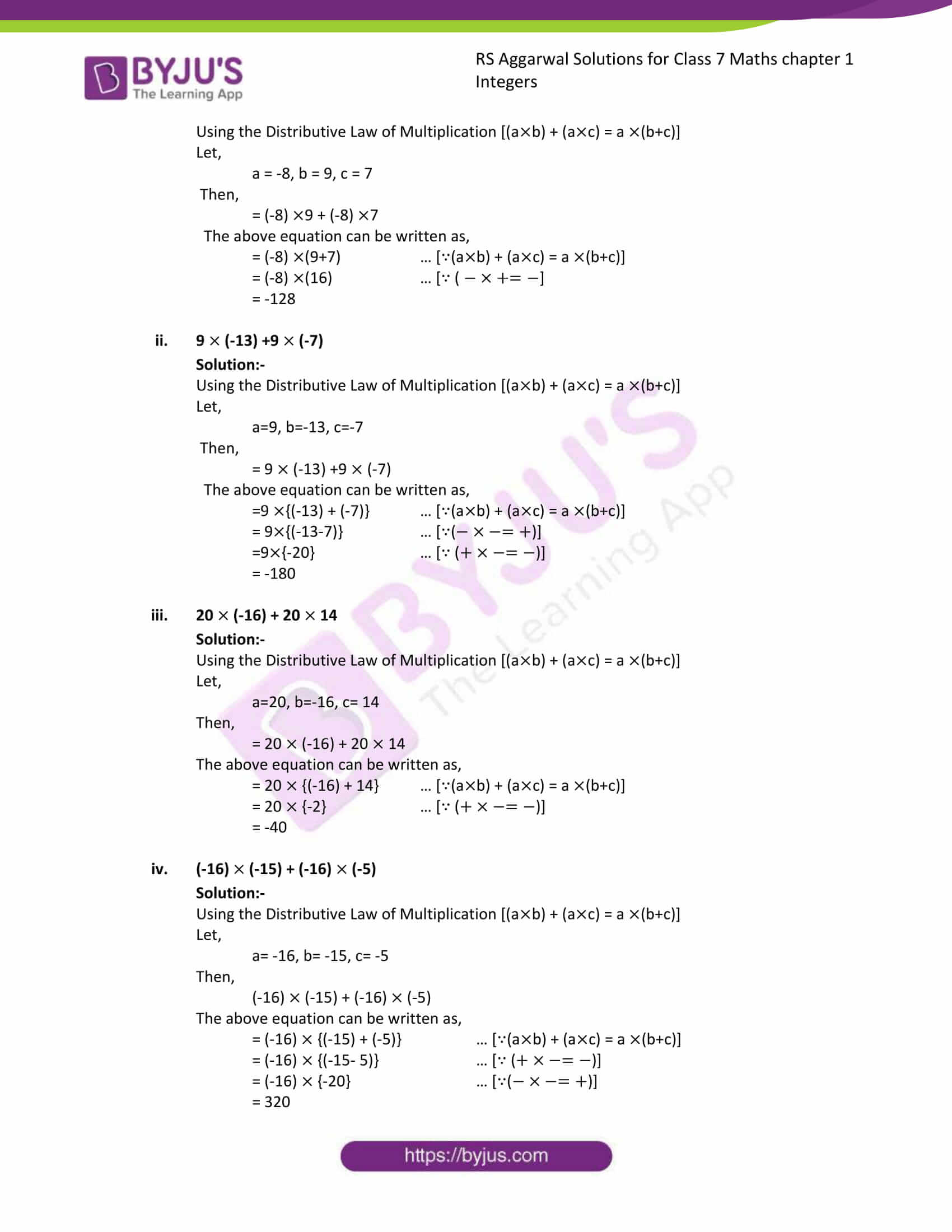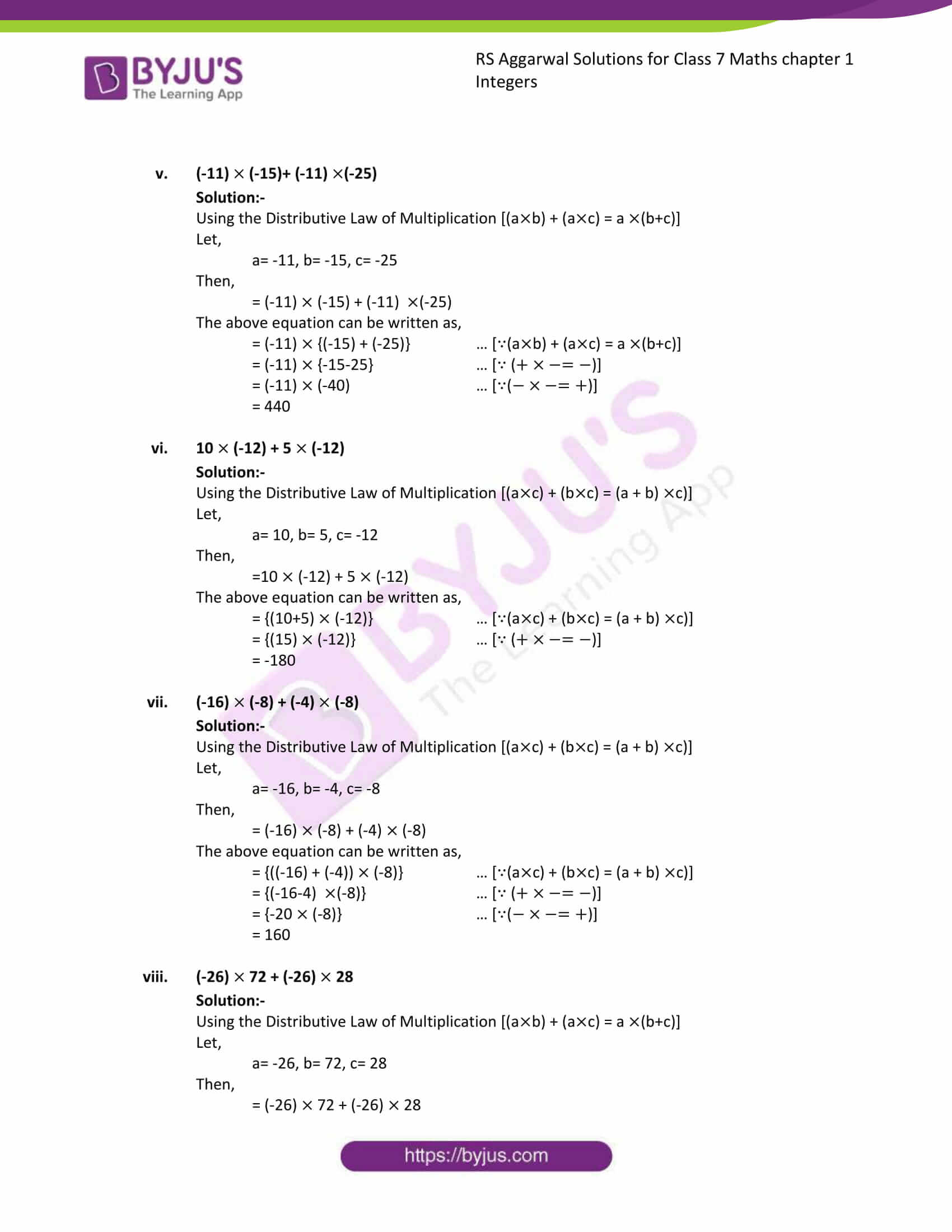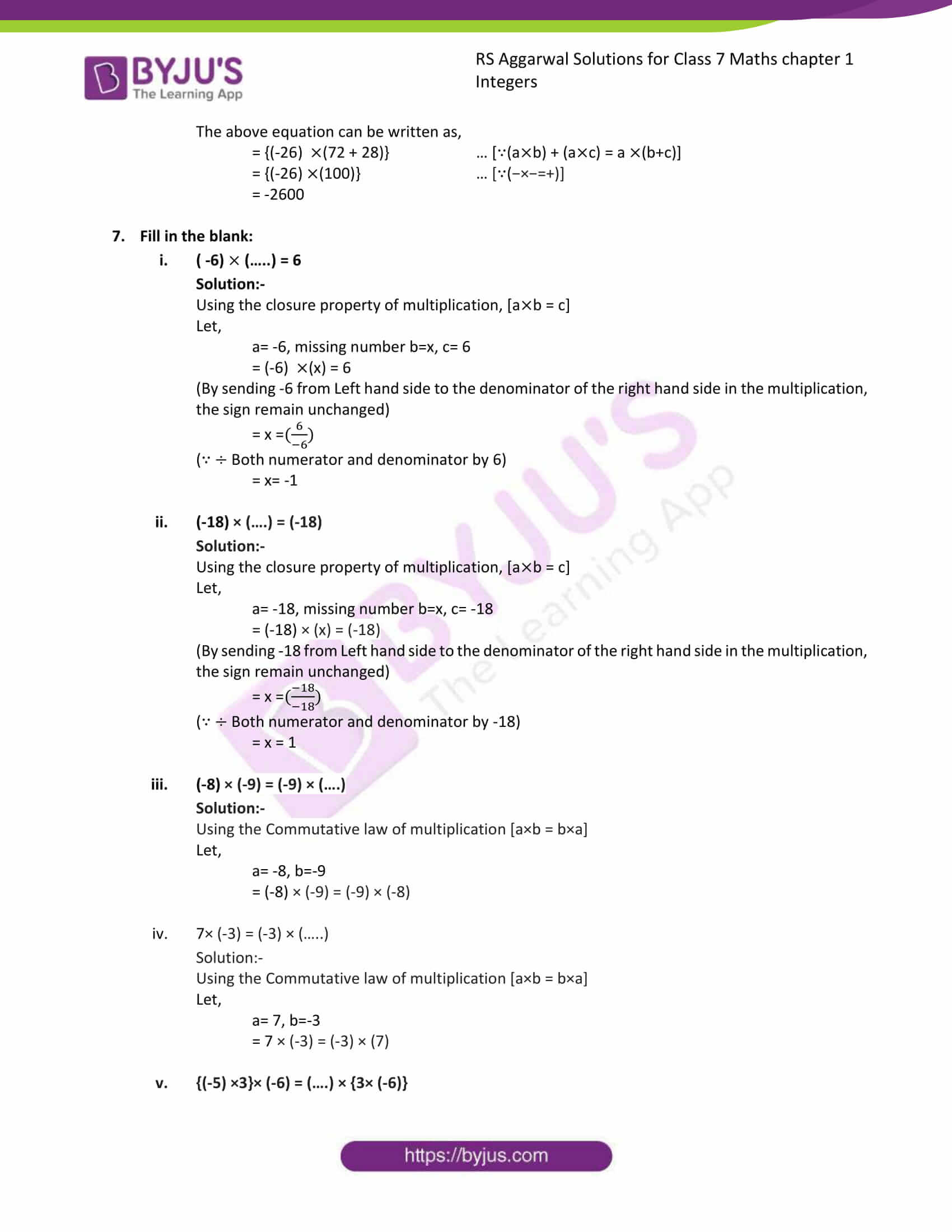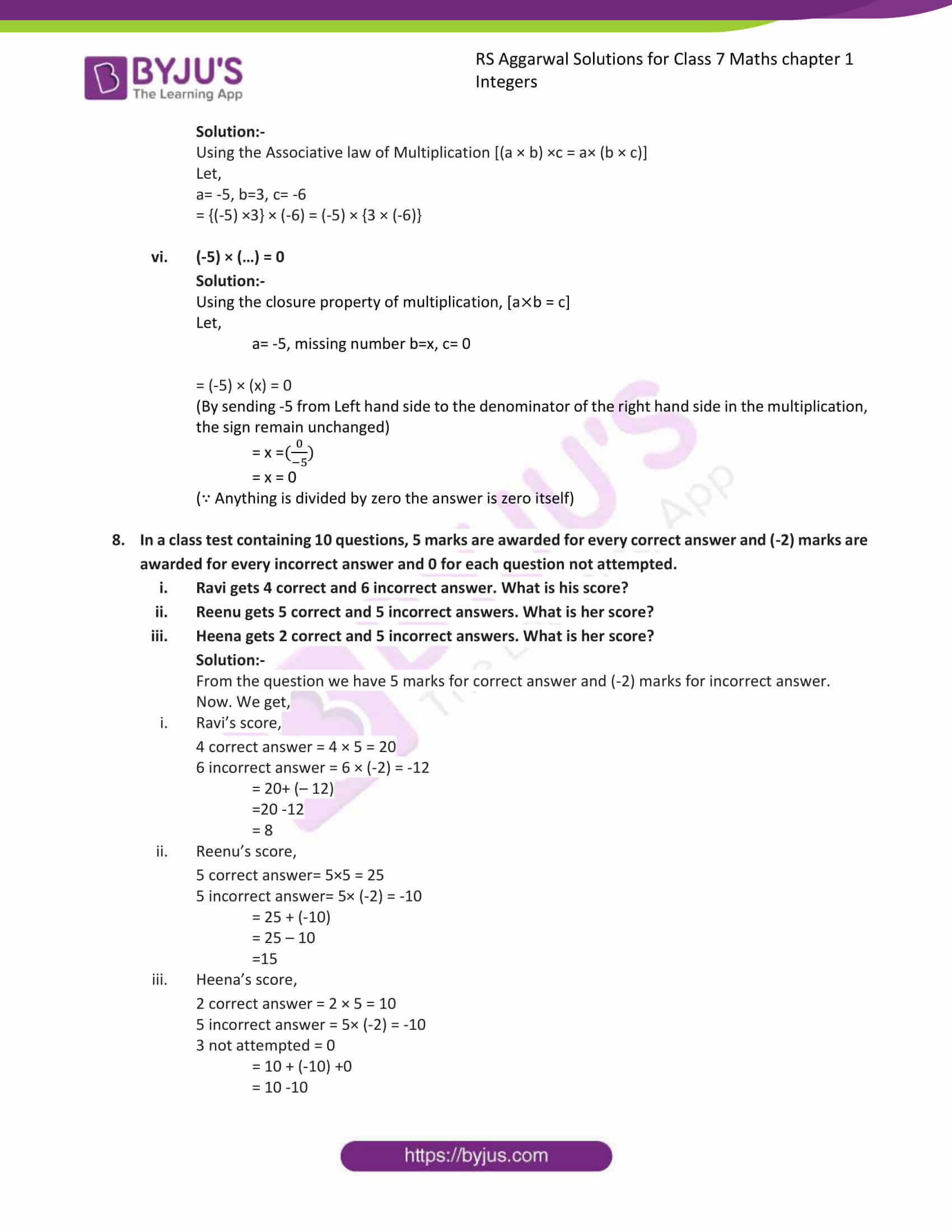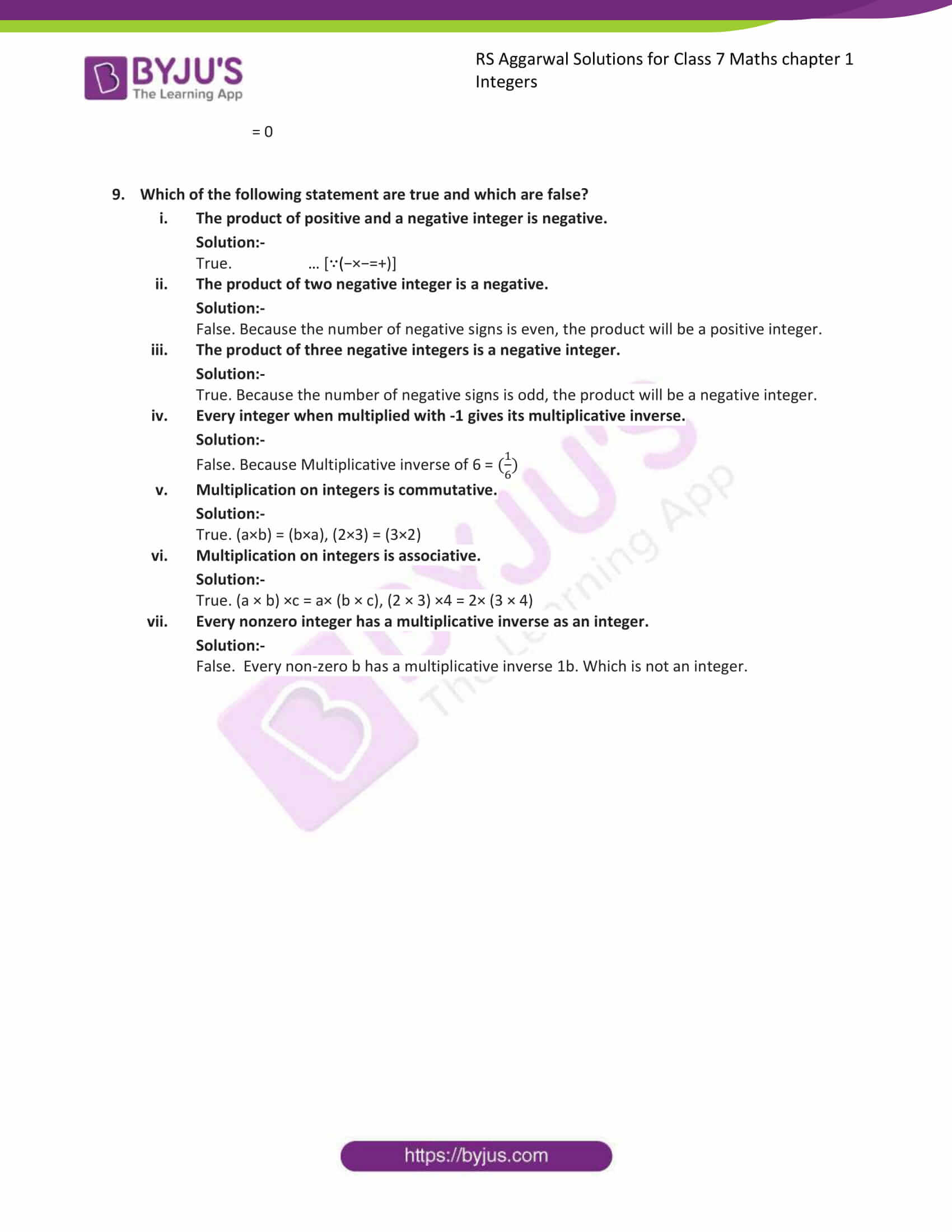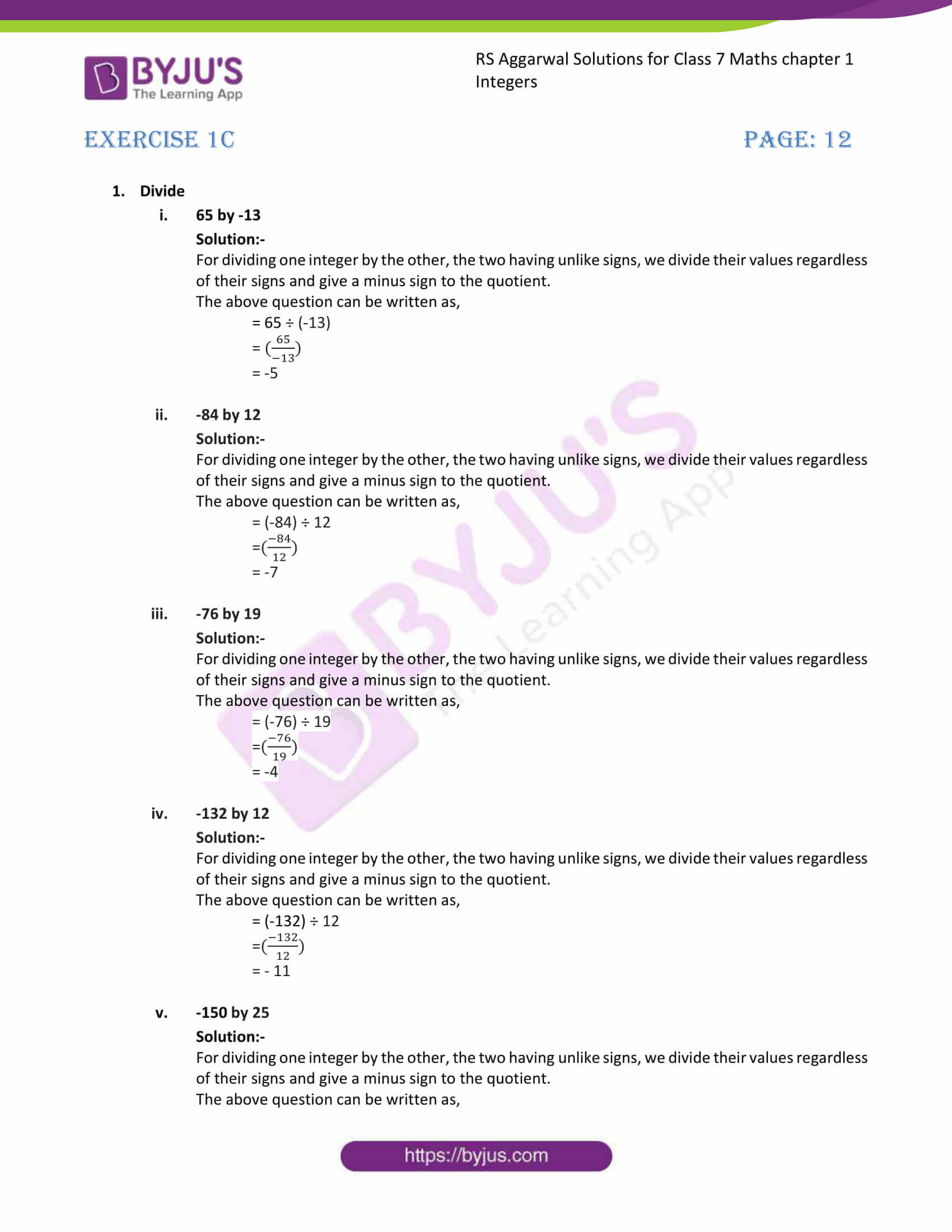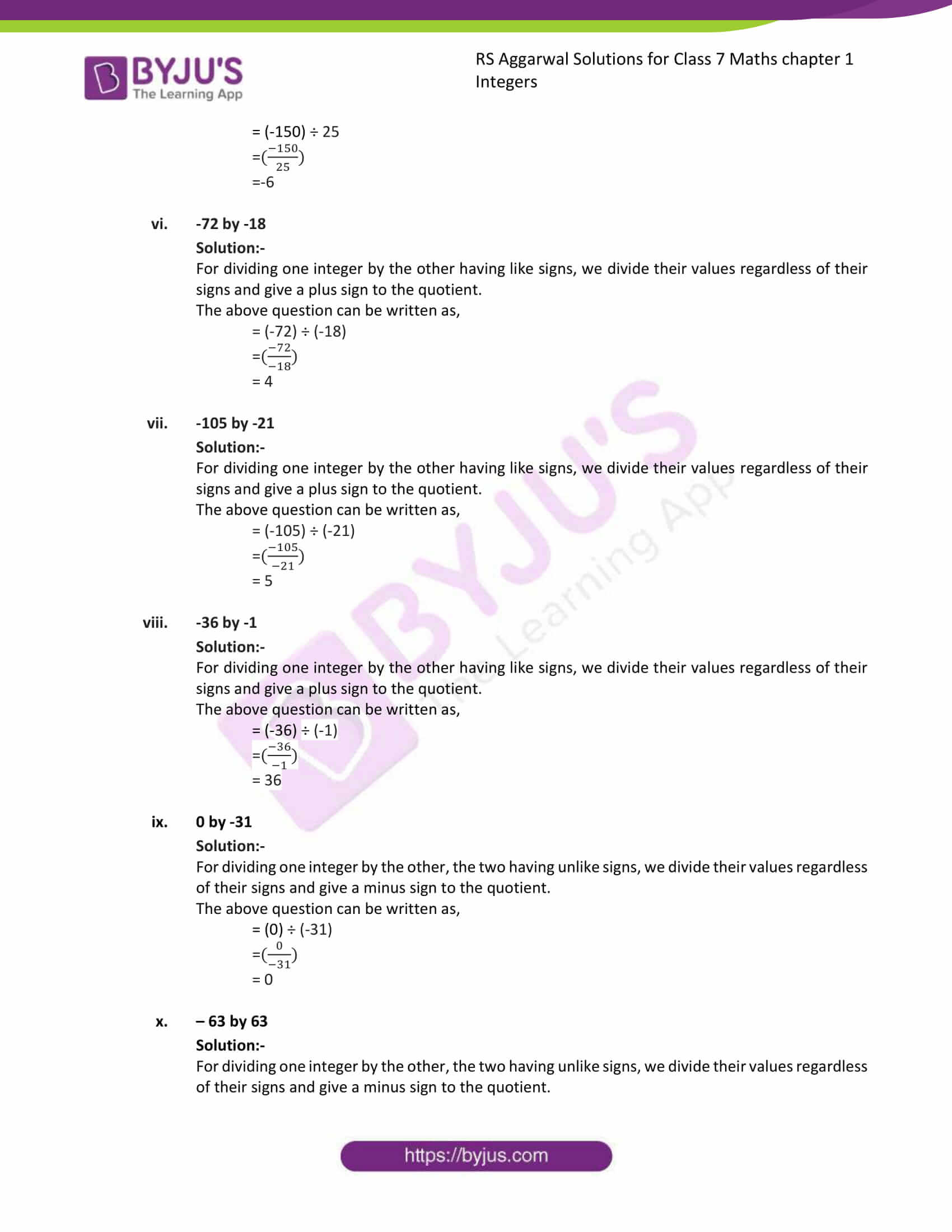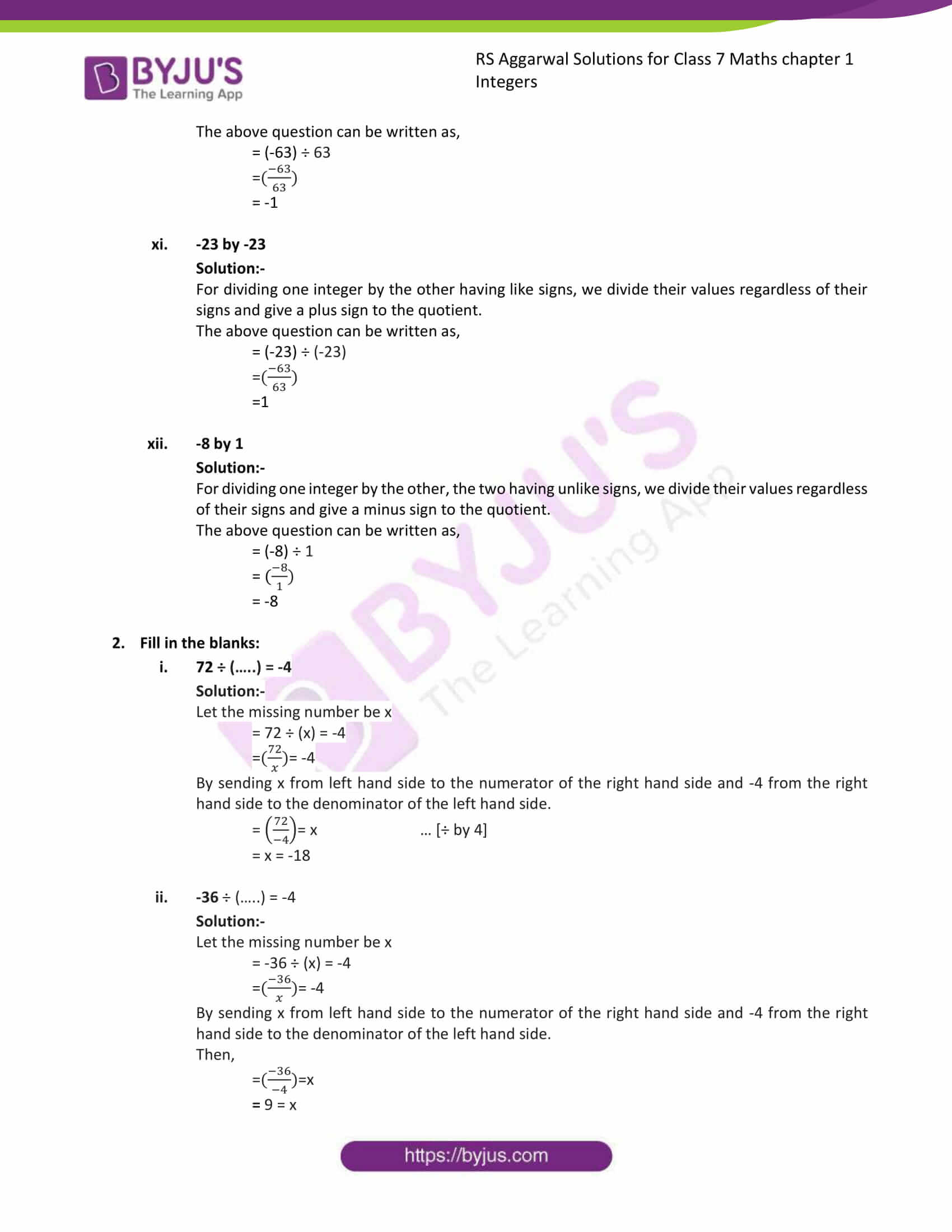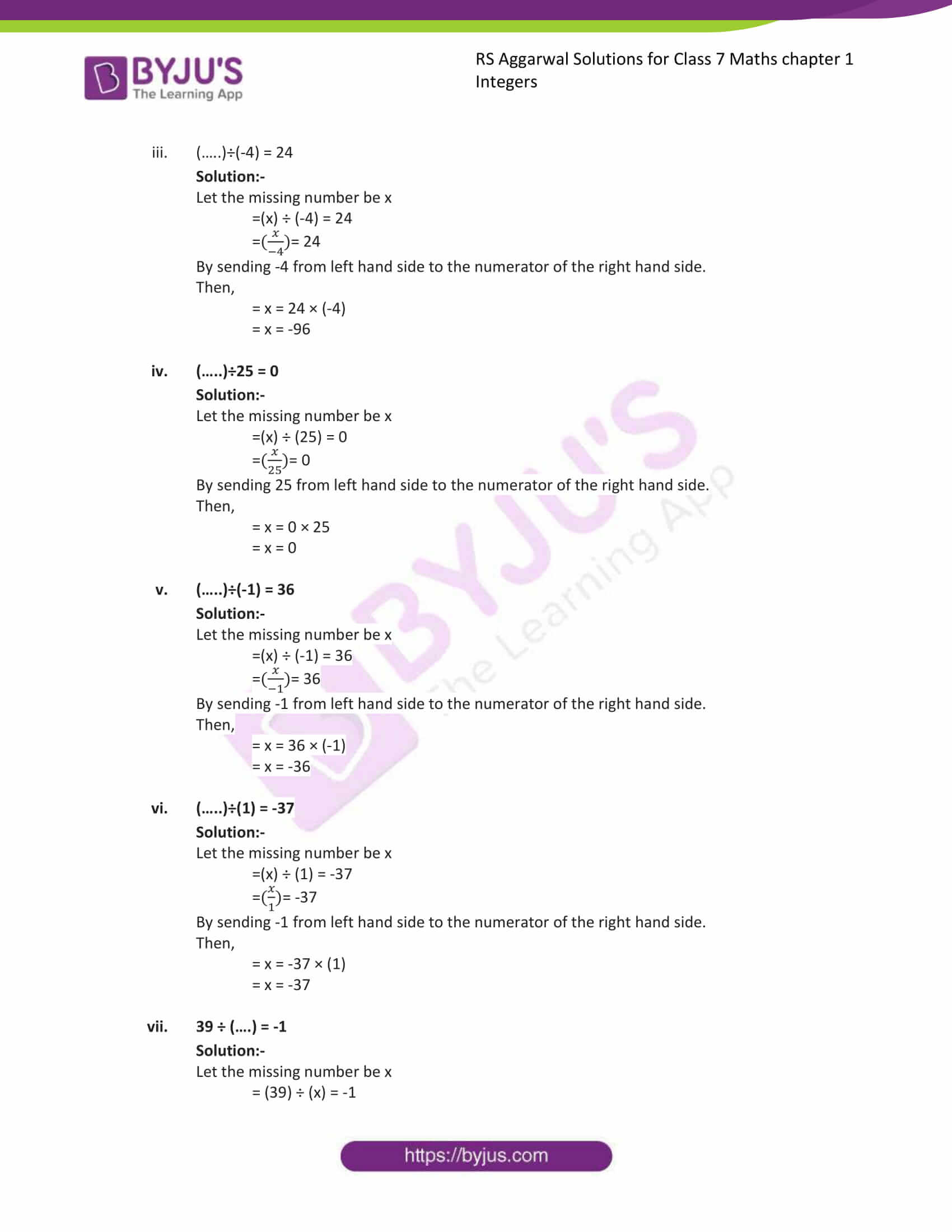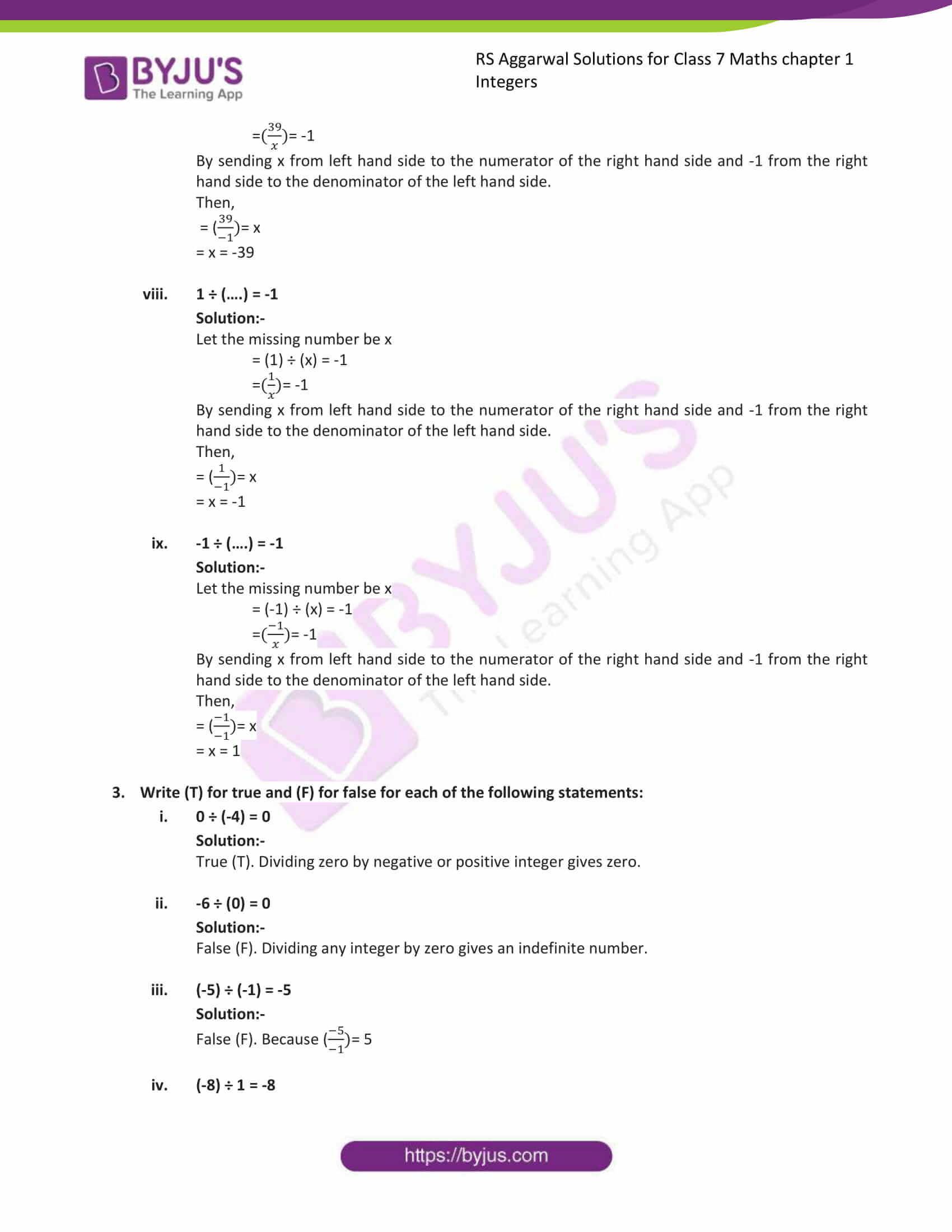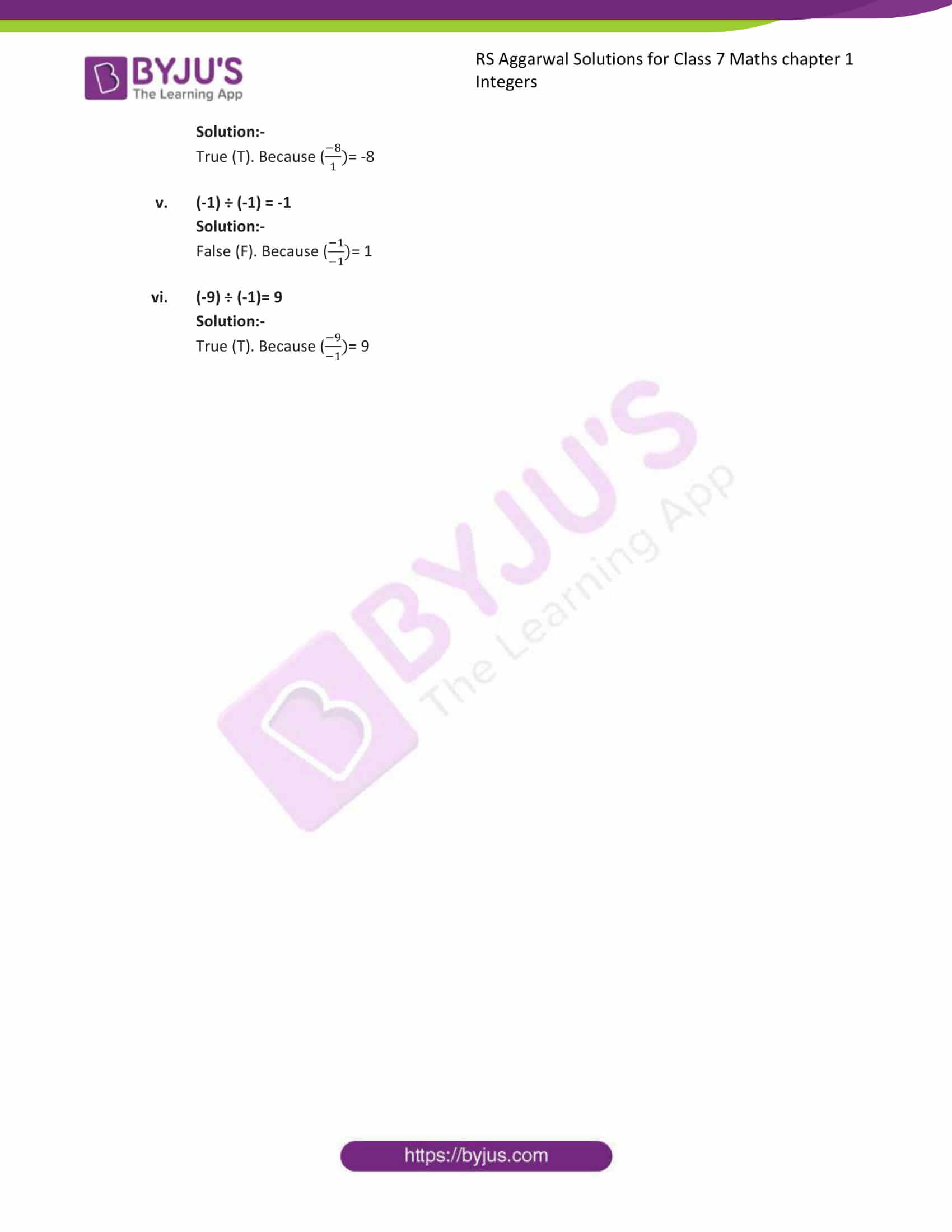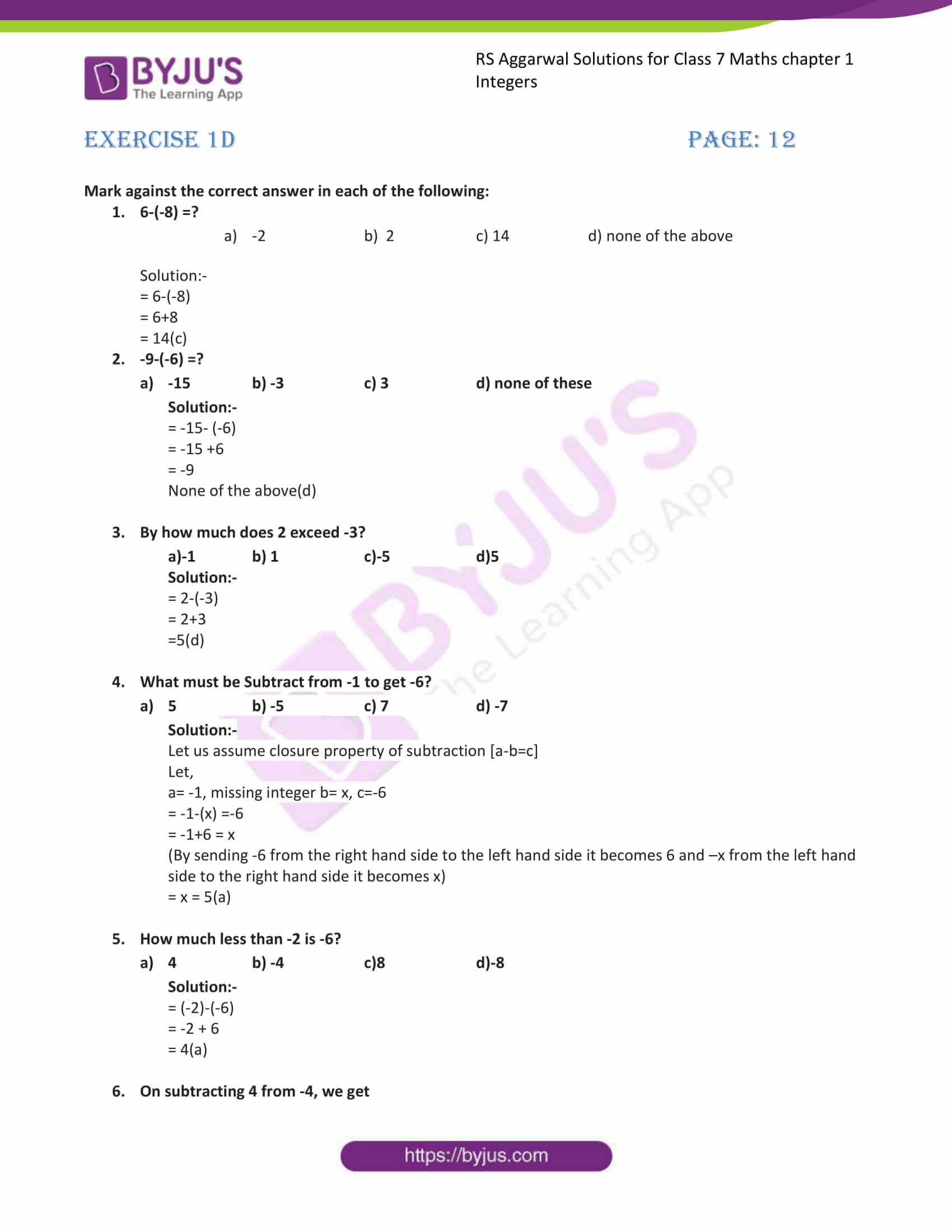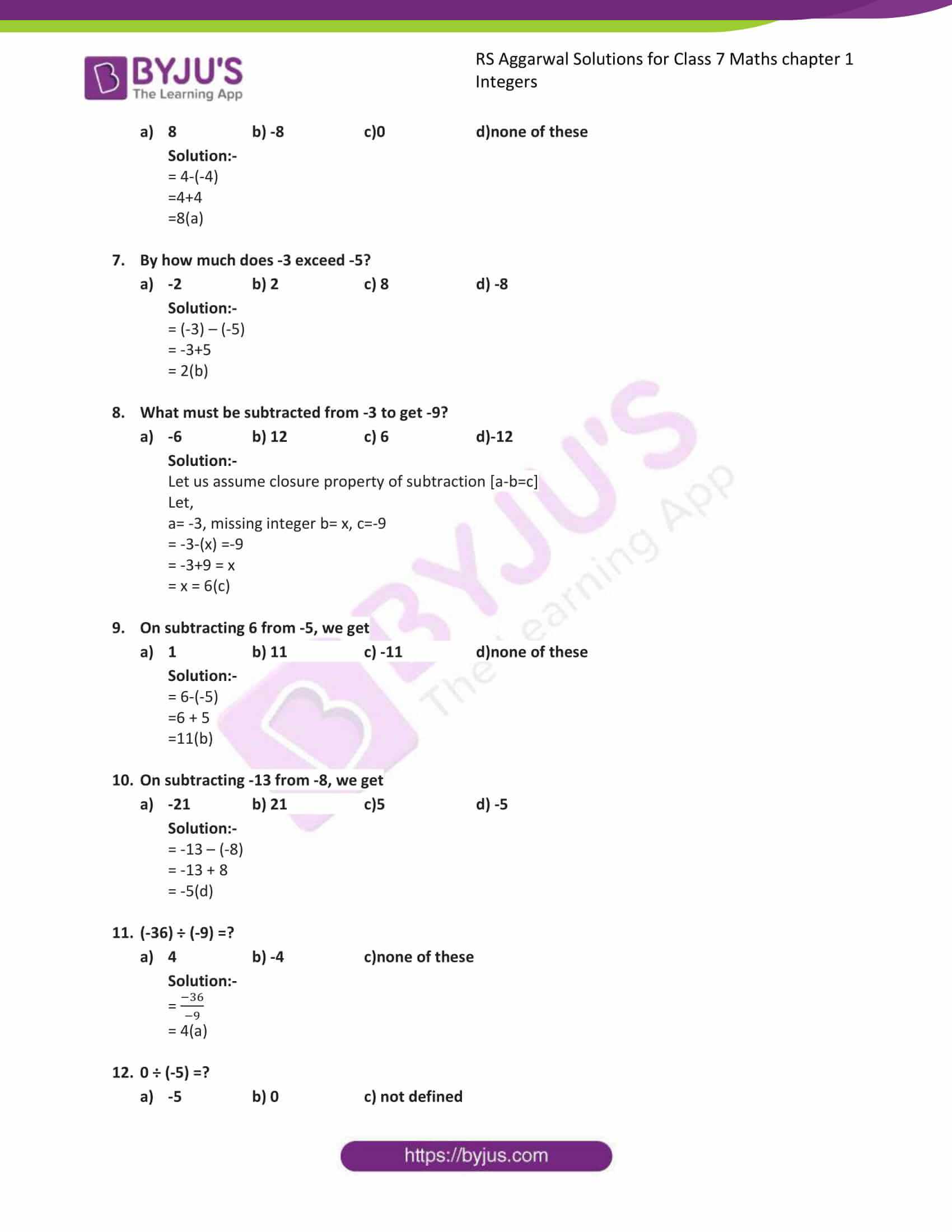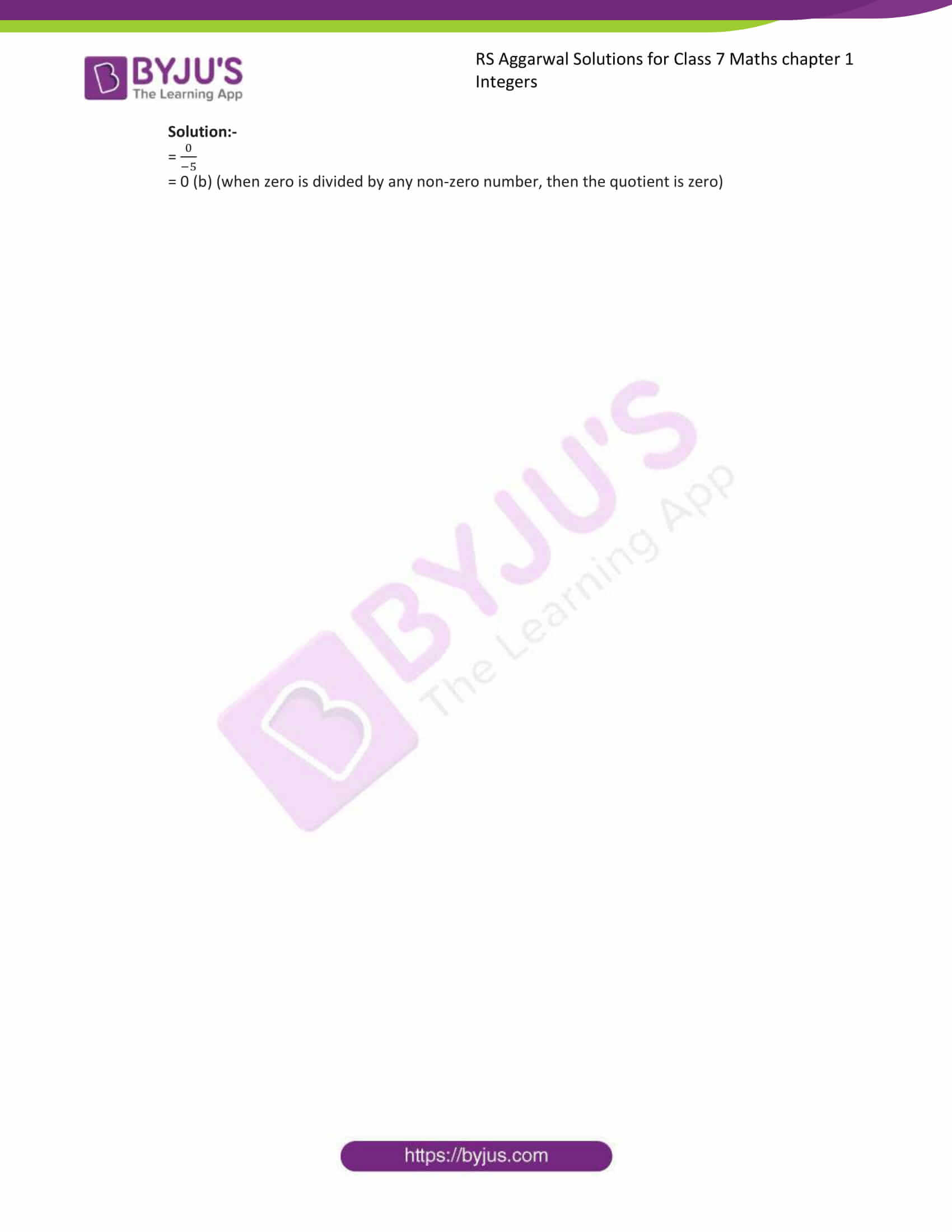Exercise 1A

Exercise 1B

Exercise 1C

Exercise 1D

## Exercise 1A

1. Evaluate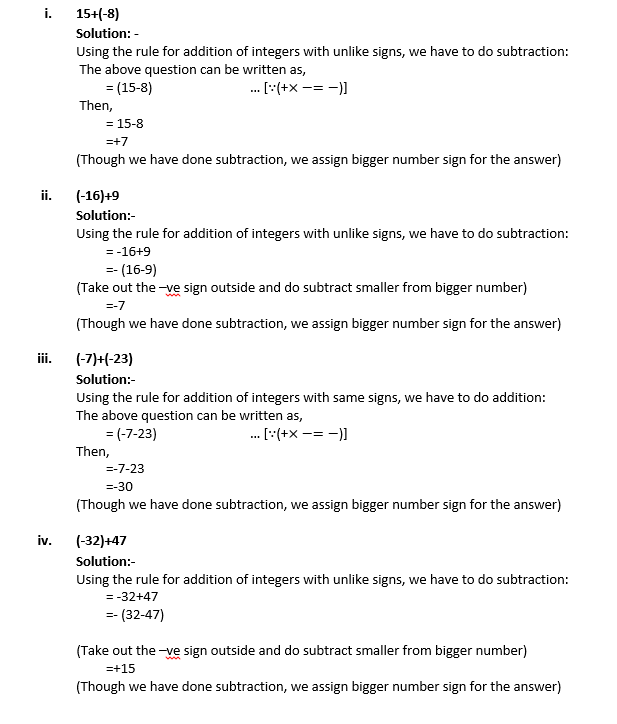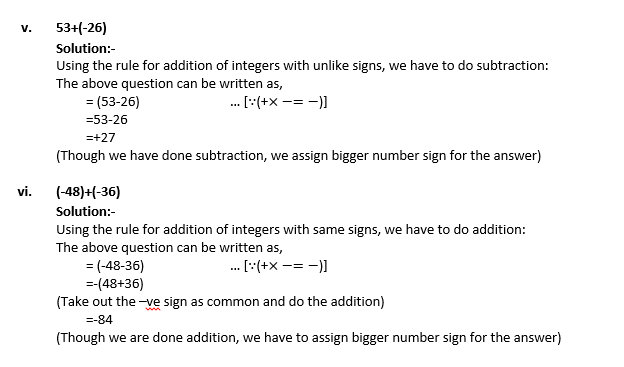2. Find the sum of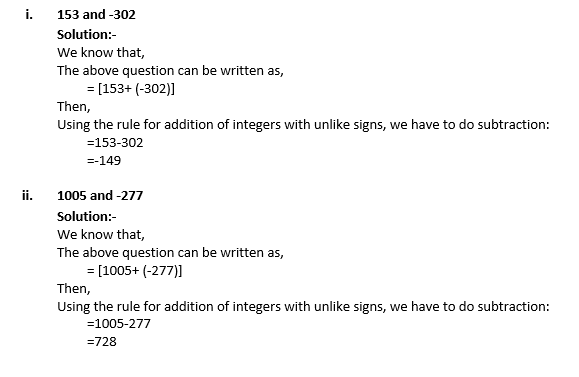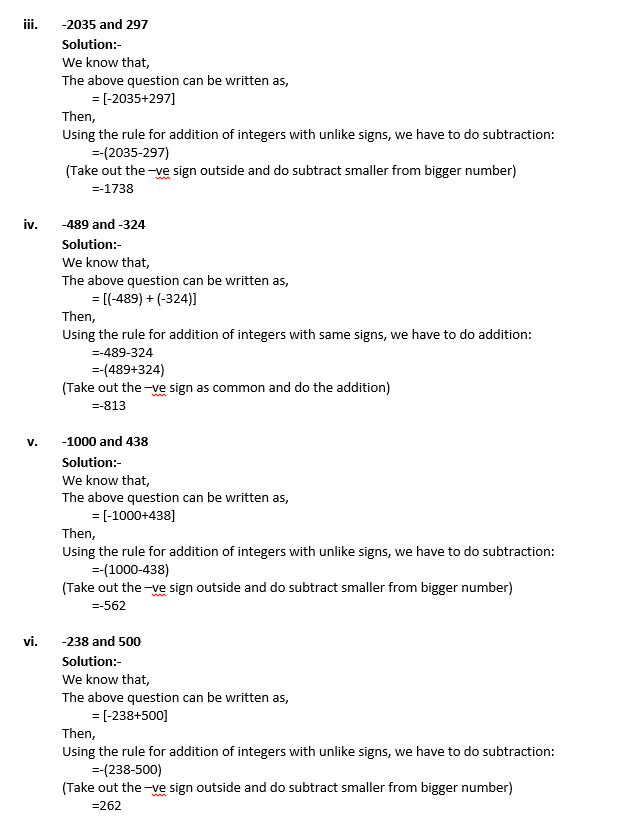3. Find the additive inverse of: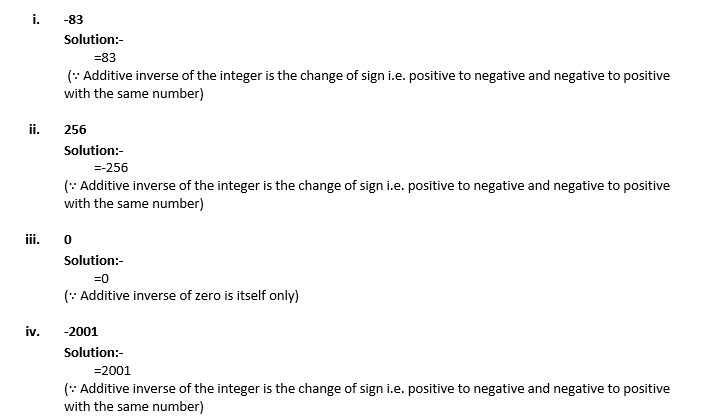4. Subtract: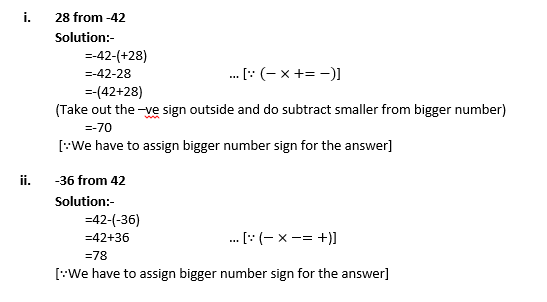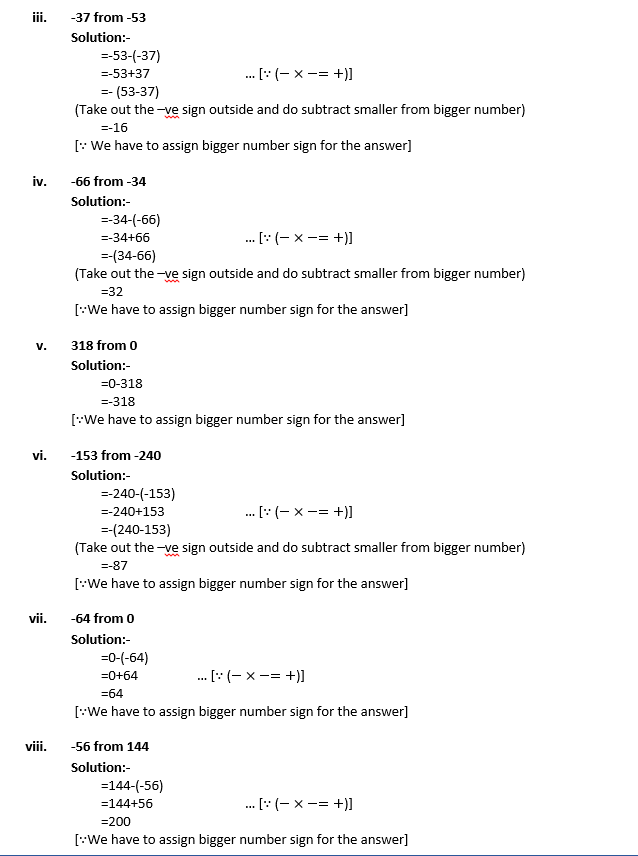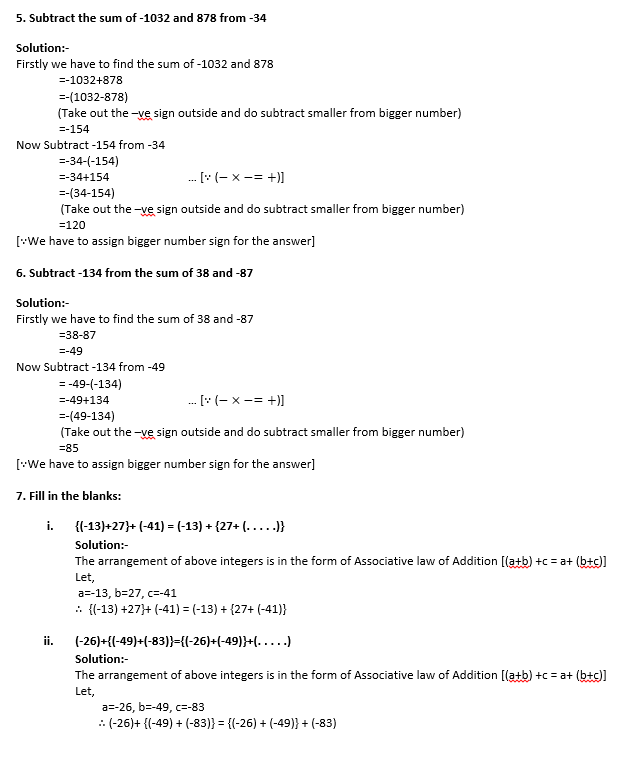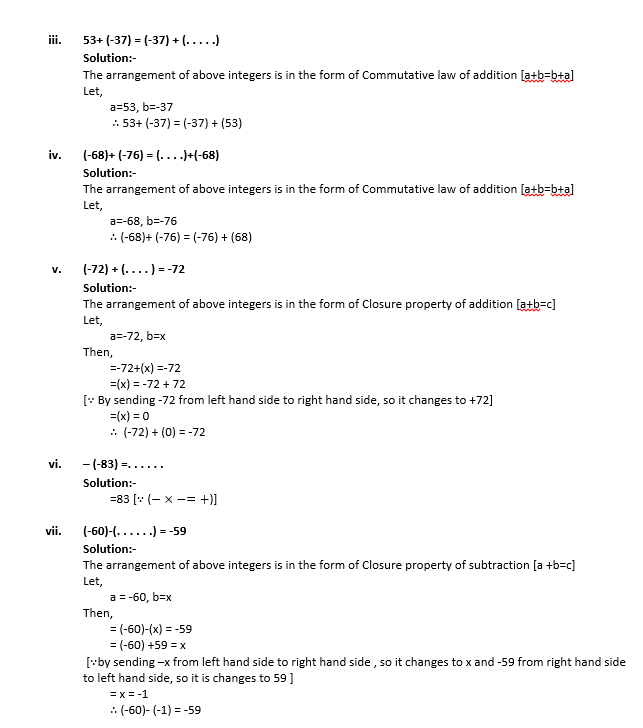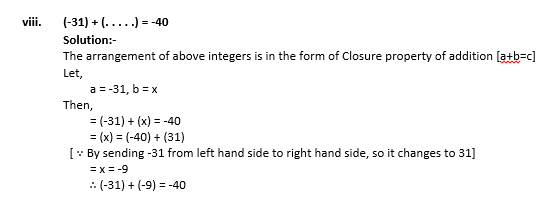8. Simplify: {-13 –(-27)}+ {-25-(-40)}

Solution:-

= {-13+27} + {-25 + 40} …[∴( – x – = + ) ]

= {14} + {15}

= 29

9. Find 36-(-64) and (-64)-36. Are they equal

Solution:-

From the commutative law of Subtraction [a-b = b-a]

Let,

a=36, b=-64

Left hand side (LHS),

= {36 – (-64)}

= {36+64}

= 100

Right hand side (RHS),

= (-64-36)

=-(64+36)

(Take out the –ve sign outside and do subtract smaller from bigger number)

=-100

By comparing LHS and RHS,

LHS ≠RHS

10. If a = -8, b = -7, c = 6, verify that (a+b) + c = a+ (b+c)

Solution:-

From the Associative law of Addition

= {-8+ (-7)} +6= -8 + {(-7) +6}

Left hand side (LHS),

= {-8+ (-7)} +6

= {-8-7} +6

= {-15} + 6

= -9

Right hand side (RHS),

=-8 + {(-7) +6}

=-8 + {-7+6}

=- 8 + {-1}

=-8-1

=-9

By comparing LHS and RHS,

LHS=RHS

11. If a=-9 and b=-6, show that (a-b) ≠ (b-a)

Solution:-

From the Commutative law of Subtraction

Left hand side (LHS),

= {(-9)-(-6)}

= {-9+6}

=-{9-6}

(Take out the –ve sign outside and do subtract smaller from bigger number)

=-{3}

=-3

Right hand side (RHS),

= {(-6)-(-9)}

= {-6+9}

=-{6-9}

(Take out the –ve sign outside and do subtract smaller from bigger number)

=-{-3}

=3

By comparing LHS and RHS

LHS≠RHS

12. The sum of two integers is -16. If one of them is 53, find the other.

Solution:-

By assuming Closure property of addition [a+b=c],

Let,

a=53, assume b=x, c=-16

=53+x=-16

= x =-16-53

( By sending 53 from left hand side to the right hand side, it changes to -53)

= x = -69

By the calculation we got another integer is -69

13. The sum of two integers is 65. If one of them is -31, find the other

Solution:-

By assuming Closure property of addition [a+b=c],

Let,

a=-31, assume b=x, c=65

= -31 + x = 65

= x = 65+31

( By sending -31 from left hand side to the right hand side, it changes to 31)

= x = 96

By the calculation we got another integer is 96

14. The difference of an integer a and (-6) is 4. Find the value of a.

Solution:-

By assuming Closure property of Subtraction [a-b=c],

Let,

a = a, b=-6, c=4

= a – (-6) = 4

= a + 6 = 4

= a = 4-6

( By sending 6 from left hand side to the right hand side, it changes to -6)

= a = -2

15. Write a pair of integers whose sum gives

(i) Zero:
(ii) A negative integer:
(iii) An integer smaller than both integers:
(iv) An integer greater then both integers:
(v) An integer smaller than only one of the integers.

Solution:-

(i) = 5 + (-5)

= 5-5

=0

(ii) = -8 + (-5)

= -8 -5

=-13

(iii) = -3 +(-6)

= -3-6

=-9

(iv) = 4 + 6

=10

(v) = 7 + (-3)

= 7 – 3

= 5

16. For each of the following statements, write (T) for true and (F) for false:

(i) The smallest integer is zero.

Solution:-

False (F),

Because all the negative integers are smaller than the zero.

(ii) -10 is greater than -7.

Solution:-

False (F),

Because in the negative integer as the number increasing, its value is decreasing.

(iii) Zero is larger than every negative integer.

Solution:-

True (T),

Because in the number line all the negative numbers are come left side of the zero.

(iv) The sum of two negative integers is a negative integer.

Solution:-

True (T),

Because, we know that for same sign we have to add and then assign the greater number sign.

(v) The sum of a negative integer and a positive integer is always a positive integer.

Solution:-

False (F),

Because we find the difference between the number and assign the bigger number sign.

## Exercise 1B

1. Multiply: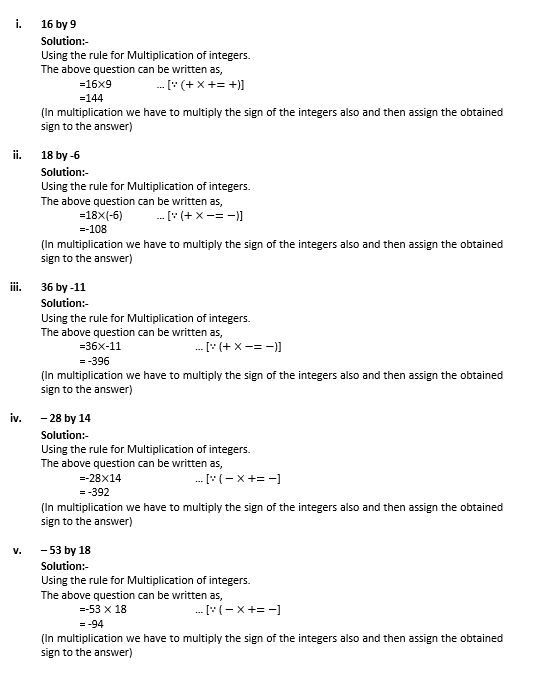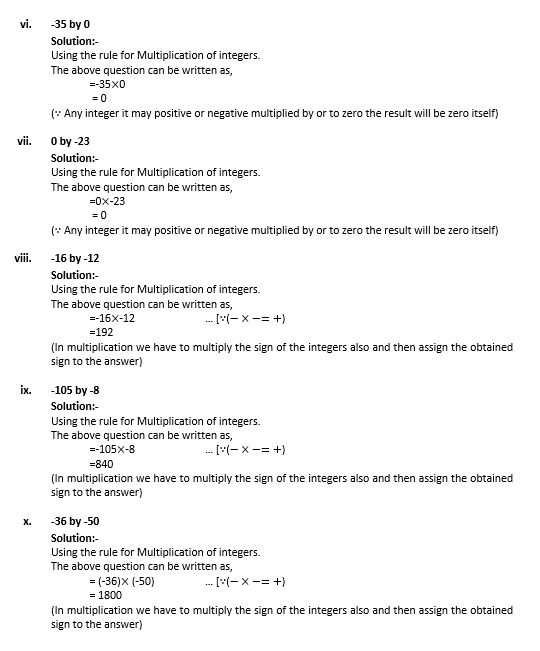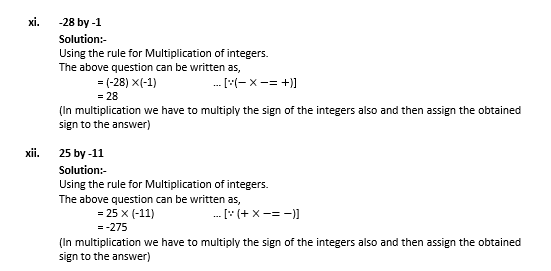1. Find each of the following products: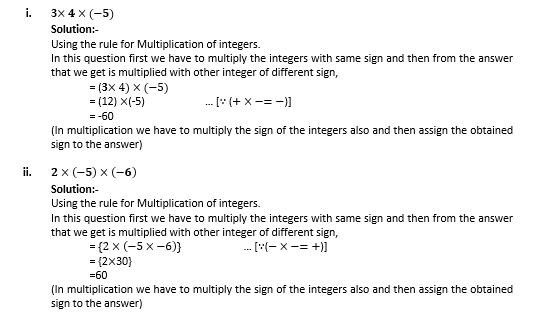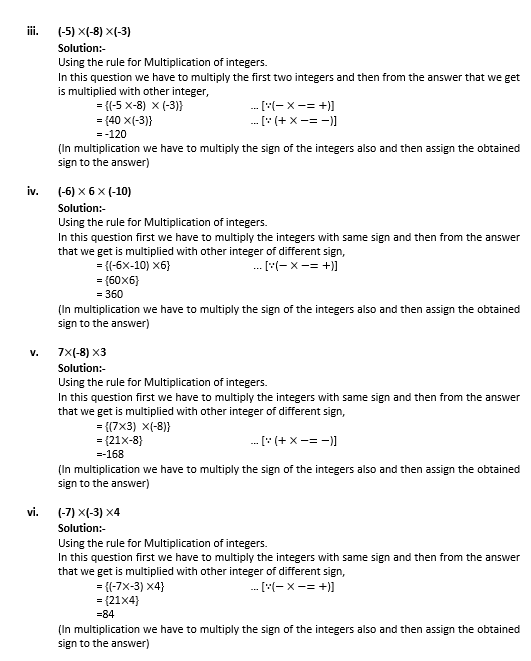2. Find each of the following products: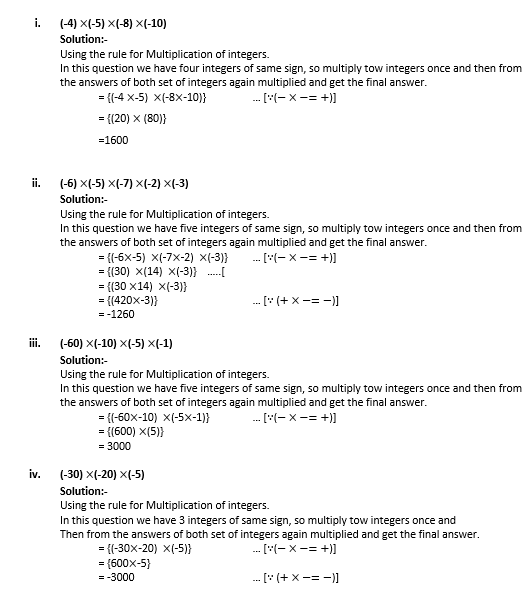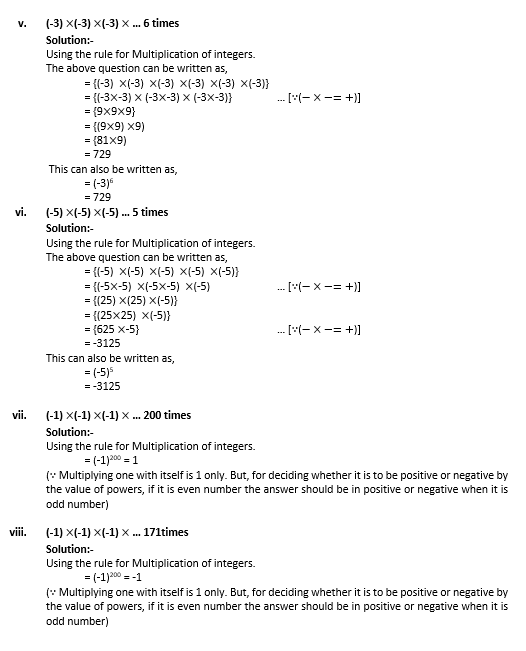4. What will be the sign of the product, if we multiply 90 negative integers and 9 positives integers?

Solution:-

If we multiply the 90 -ve integers the answer we are getting is positive integer. Because, 90 is an even number and the product of even number of negative integer is positive. The product of number of positive integer is not affect the sign of product. So the product obtained from this is positive.

5. What will be the sign of the product, if we multiply 103 negative integers and 65 positives integers?

Solution:-

If we multiply the 103 -ve integers the answer we are getting is negative integer. Because, 103 is an odd number and the product of odd number of negative integer is negative. The product of number of positive integer is not affect the sign of product. So the product obtained from this is negative.

6. Simplify: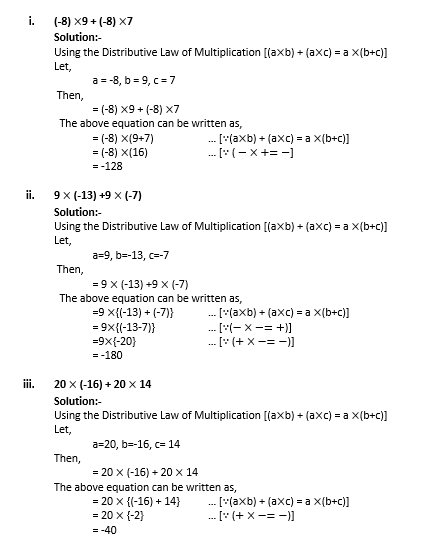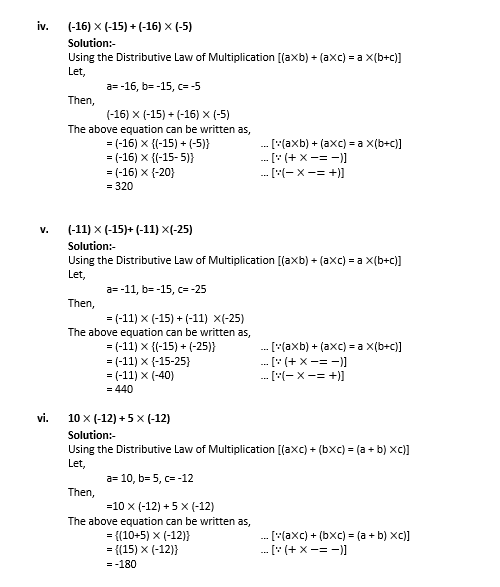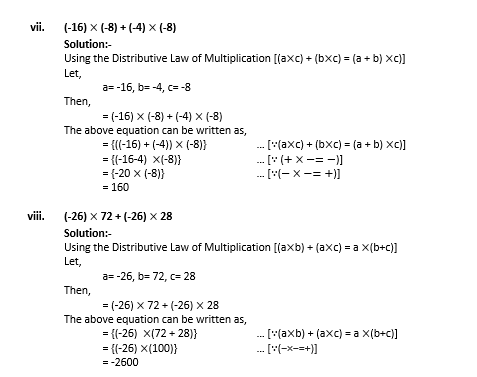7. Fill in the blank: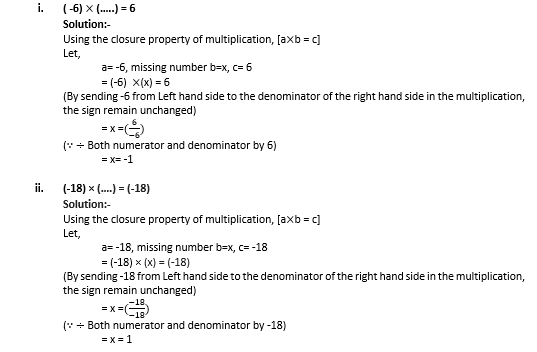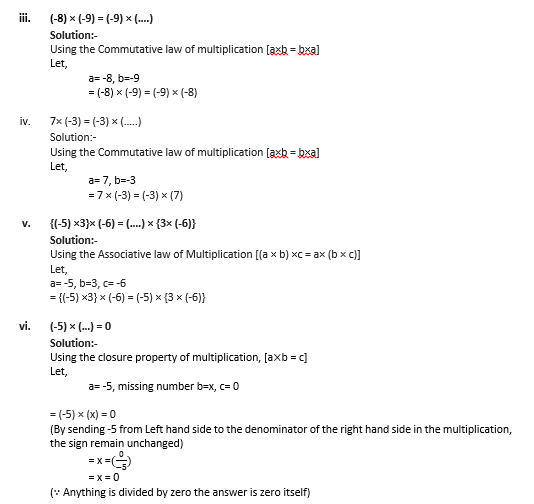8. In a class test containing 10 questions, 5 marks are awarded for every correct answer and (-2) marks are awarded for every incorrect answer and 0 for each question not attempted.
(i) Ravi gets 4 correct and 6 incorrect answer. What is his score?
(ii) Reenu gets 5 correct and 5 incorrect answers. What is her score?
(iii) Heena gets 2 correct and 5 incorrect answers. What is her score?

Solution:-

From the question we have 5 marks for correct answer and (-2) marks for incorrect answer.

Now. We get,

(i) Ravi’s score,

4 correct answer = 4 × 5 = 20

6 incorrect answer = 6 × (-2) = -12

= 20+ (– 12)

= 20 – 12

= 8

(ii) Reenu’s score,

5 correct answer= 5×5 = 25

5 incorrect answer= 5× (-2) = -10

= 25 + (-10)

= 25 – 10

=15

(iii) Heena’s score,

2 correct answer = 2 × 5 = 10

5 incorrect answer = 5× (-2) = -10

3 not attempted = 0

= 10 + (-10) +0

= 10 -10

= 0

9. Which of the following statement are true and which are false?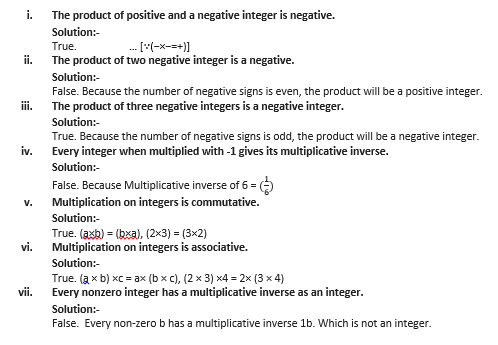## Exercise 1c

1. Divide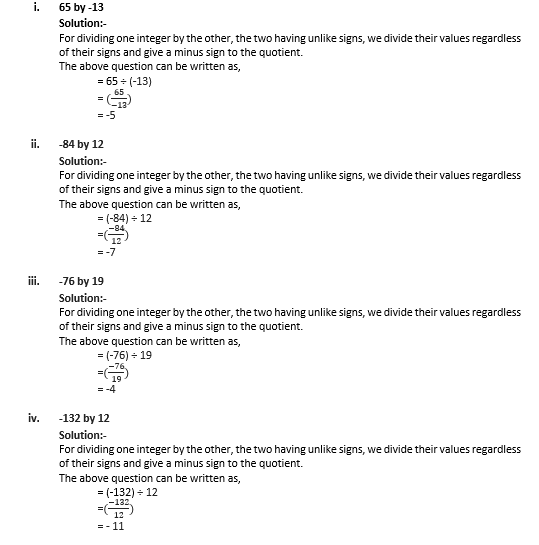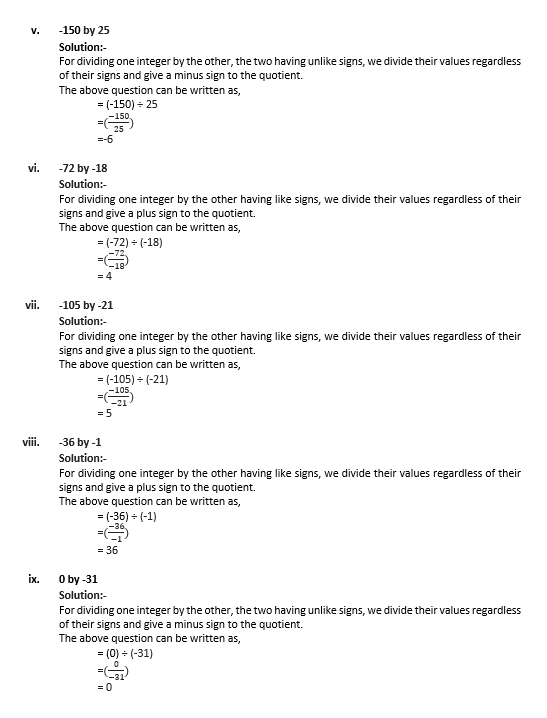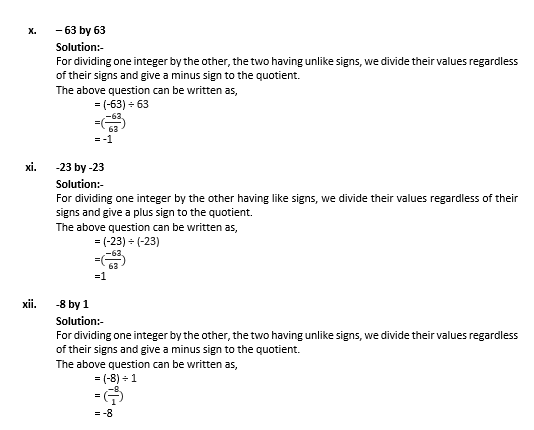2. Fill in the blanks: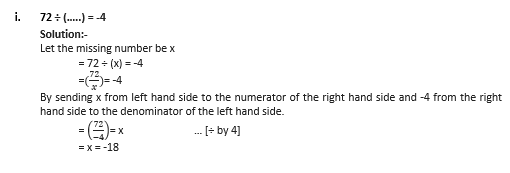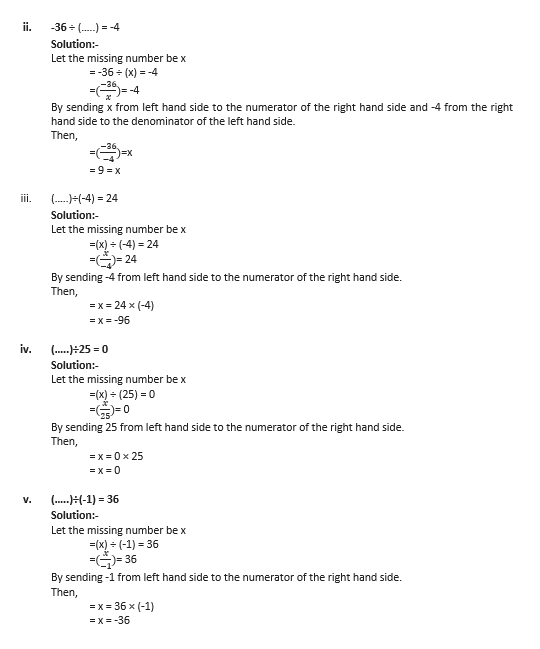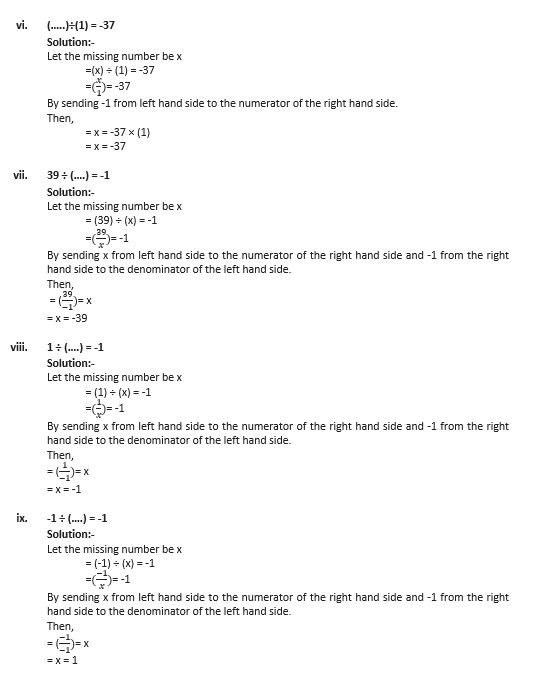3. Write (T) for true and (F) for false for each of the following statements: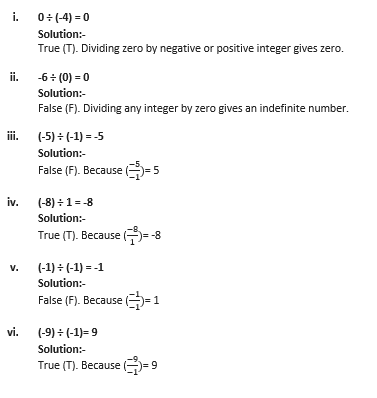## Exercise 1D Page: 12

Mark against the correct answer in each of the following: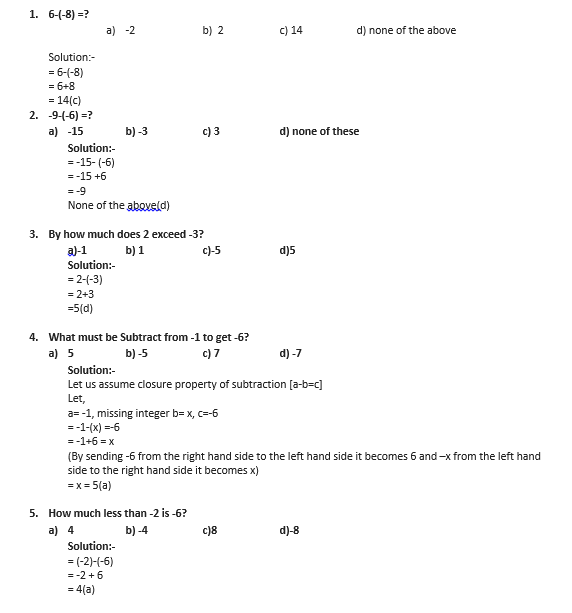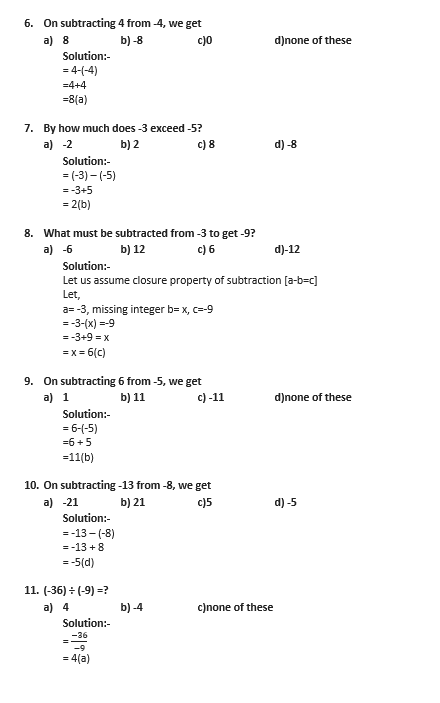12. 0 ÷ (-5) =?

a) -5 b) 0 c) not defined

Solution:-

= 0/(-5)

= 0 (b) (when zero is divided by any non-zero number, then the quotient is zero)

## RS Aggarwal Solutions for Class 7 Maths Chapter 1 – Integers

Chapter 1 – Integers contains 4 exercises and the RS Aggarwal Solutions available on this page provide solutions to the questions present in the exercises. Now, let us have a look at some of the concepts discussed in this chapter.